# Tamil Nadu SSLC Board (TN SSLC) Question Paper for Class 10th Maths 2018 In PDF

Tamil Nadu SSLC (Class 10th) Maths 2018 question paper with solutions are available in a pdf downloadable format at BYJU’S. These will help the students to access easily and practice to excel their preparation for the exam. They can also get the TN SSLC Class 10 previous year question papers along with the solutions. These solutions are given by the expert faculty at BYJU’S hence they are accurate. Students are advised to practise this Tamil Nadu Board SSLC Class 10 Maths Previous Year Question Paper 2018 regularly to get used to answering questions of various difficulty level.

Students can also download model papers of Class 10 Maths Tamilnadu Board to practice the expected questions and to know the pattern of the paper. All these papers are provided in pdf form, which can be downloaded at any time.

### Tamil Nadu Board SSLC Class 10 Maths 2018 Question Paper with Solutions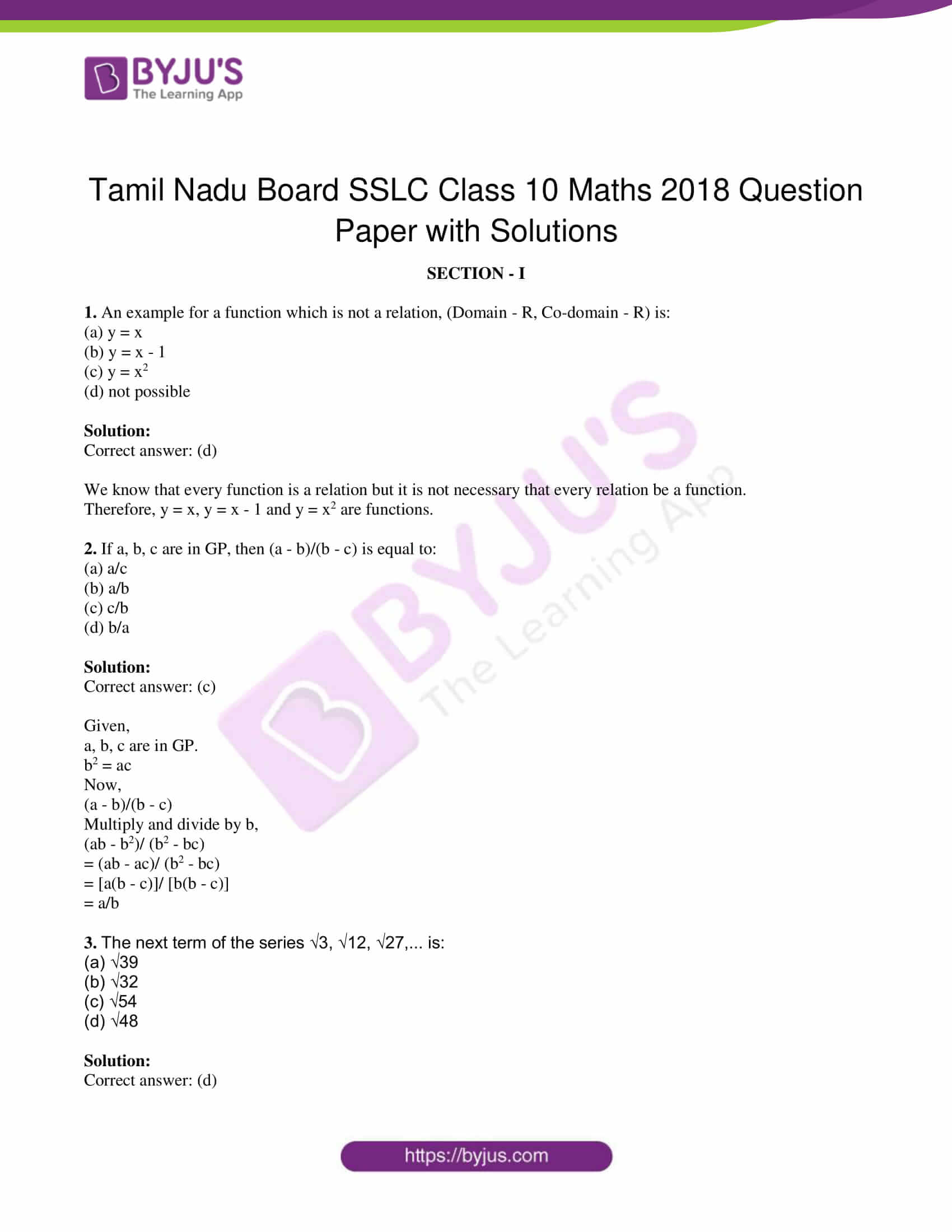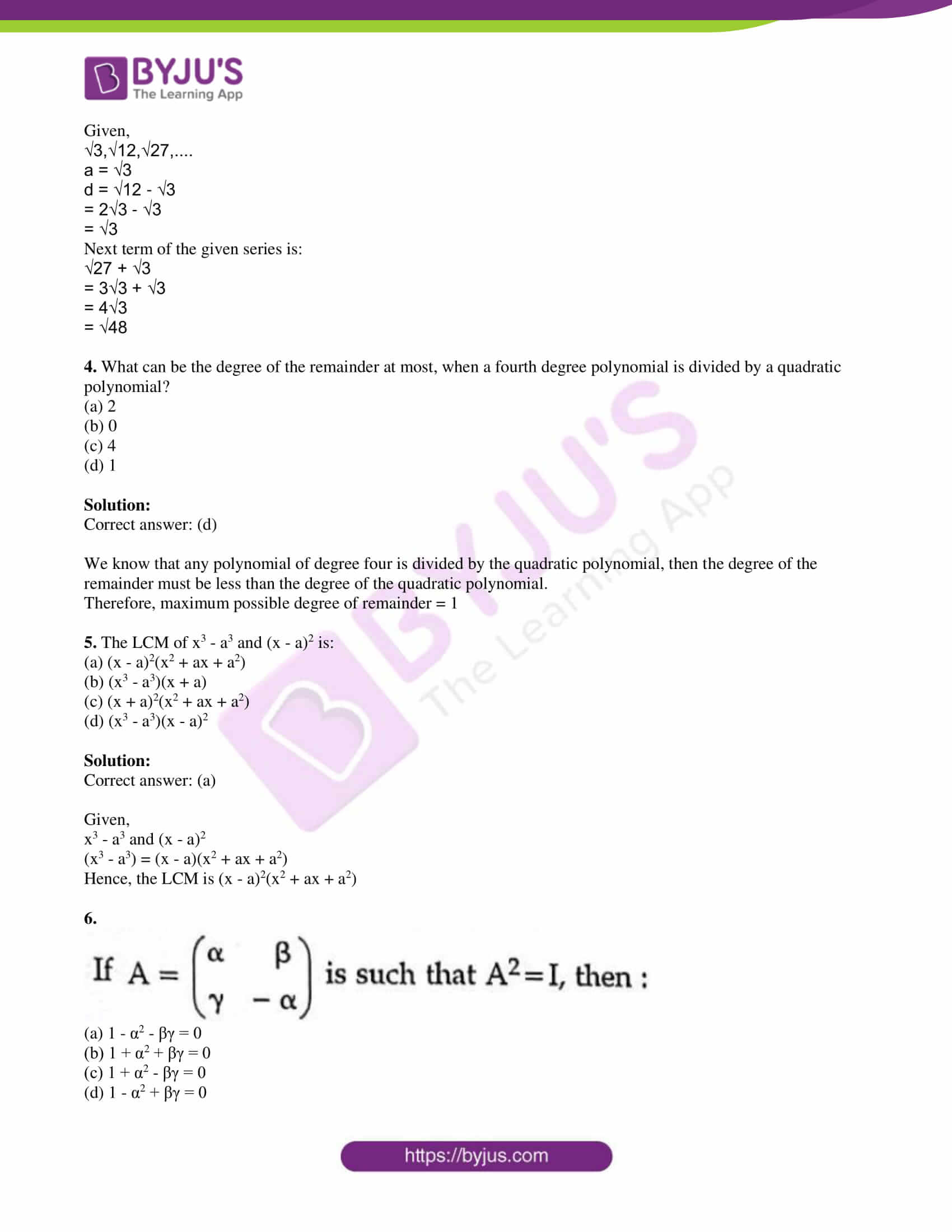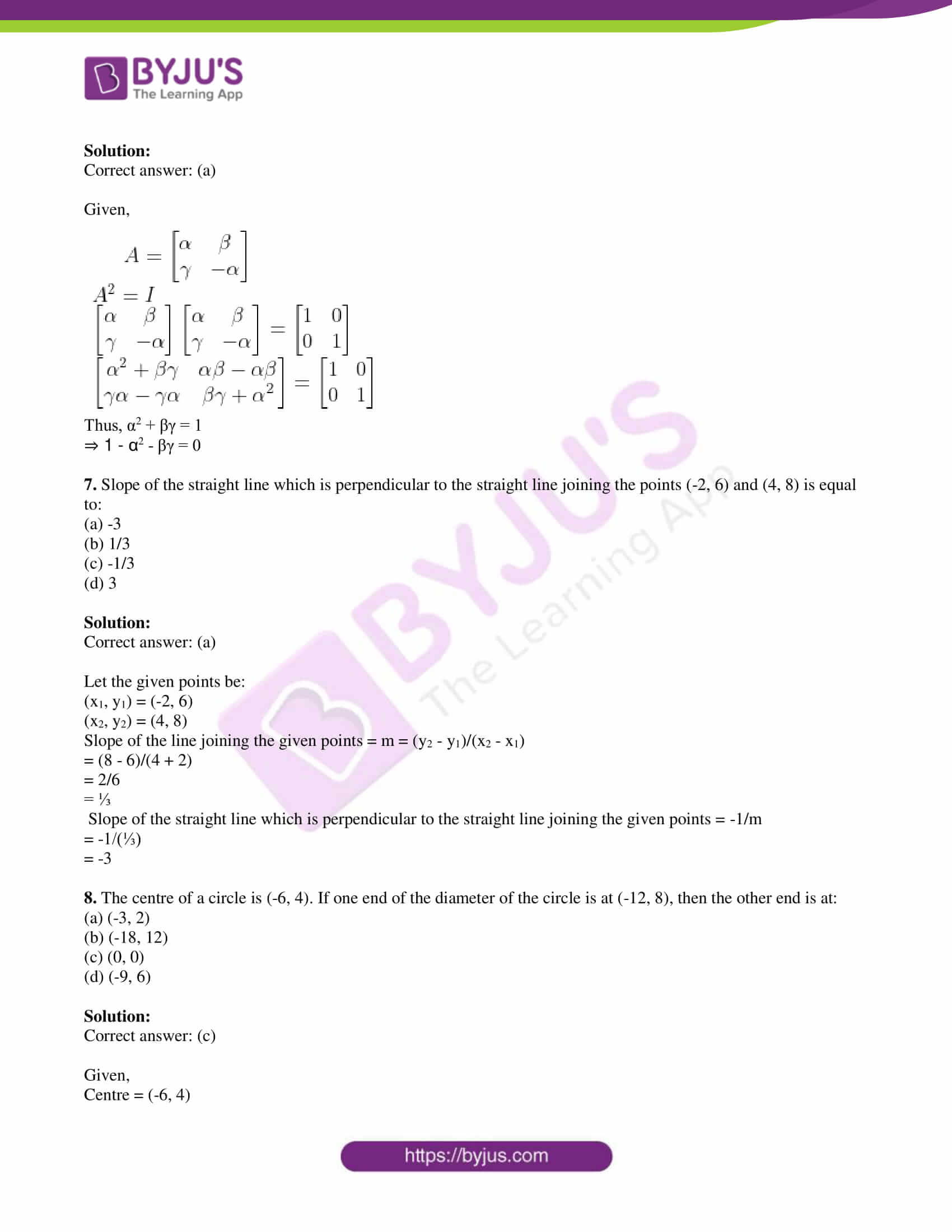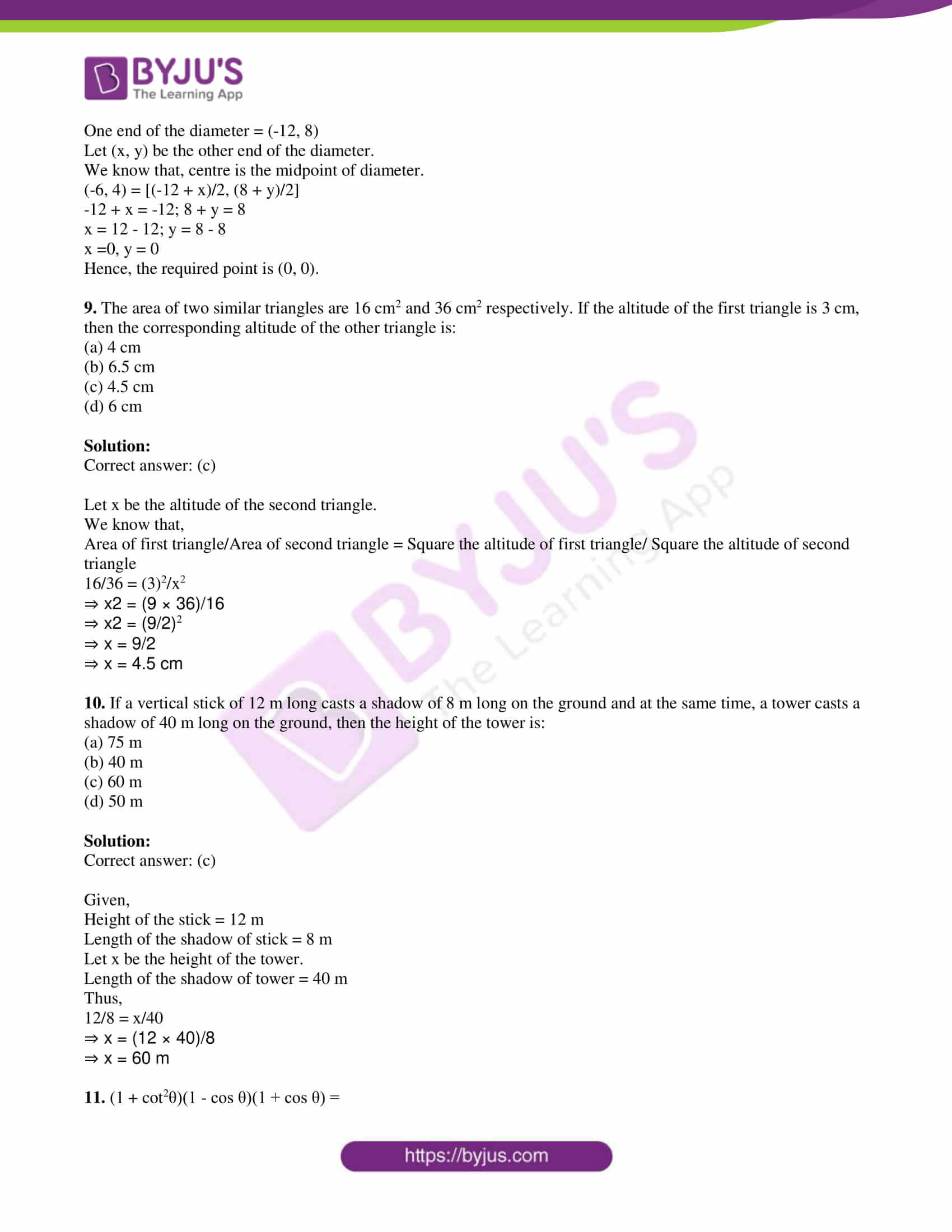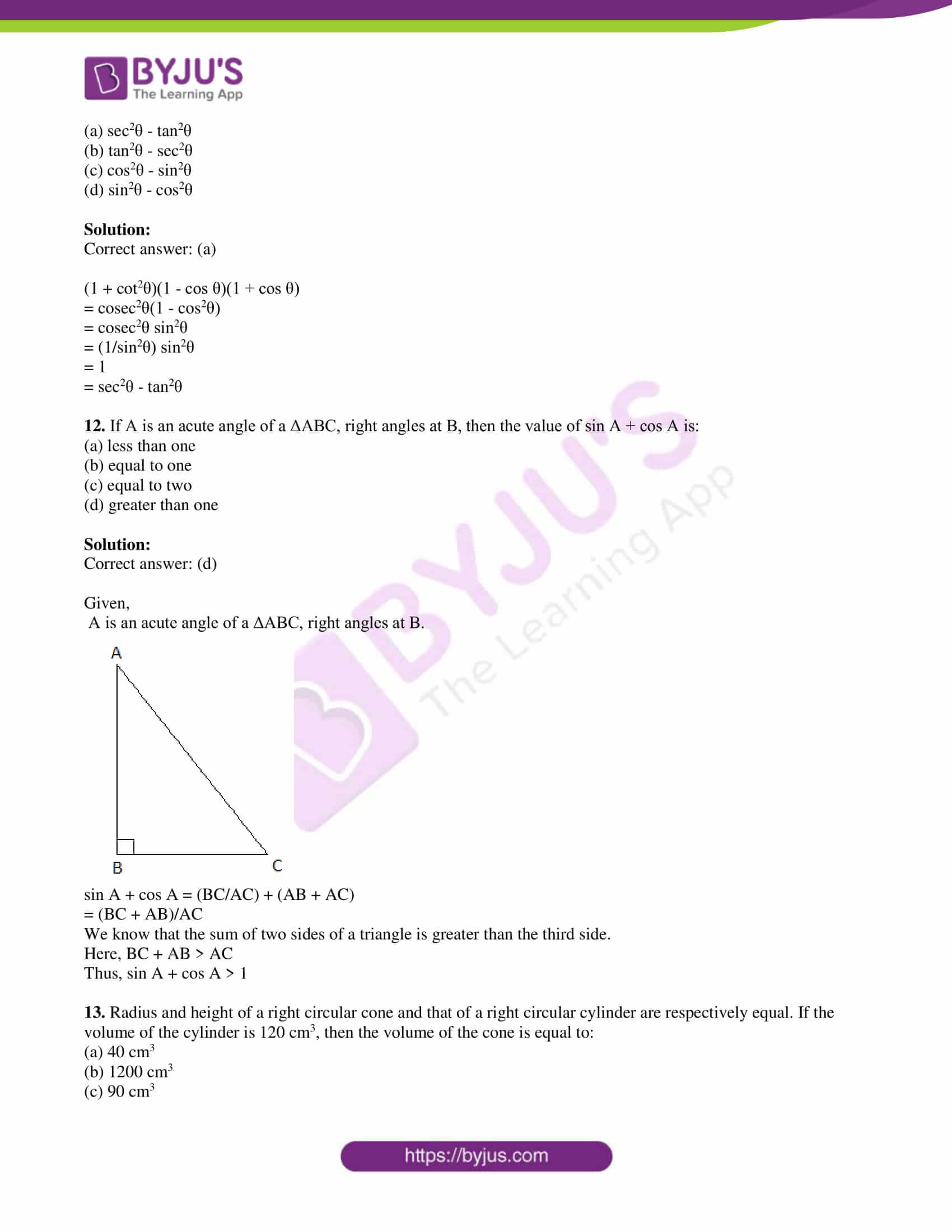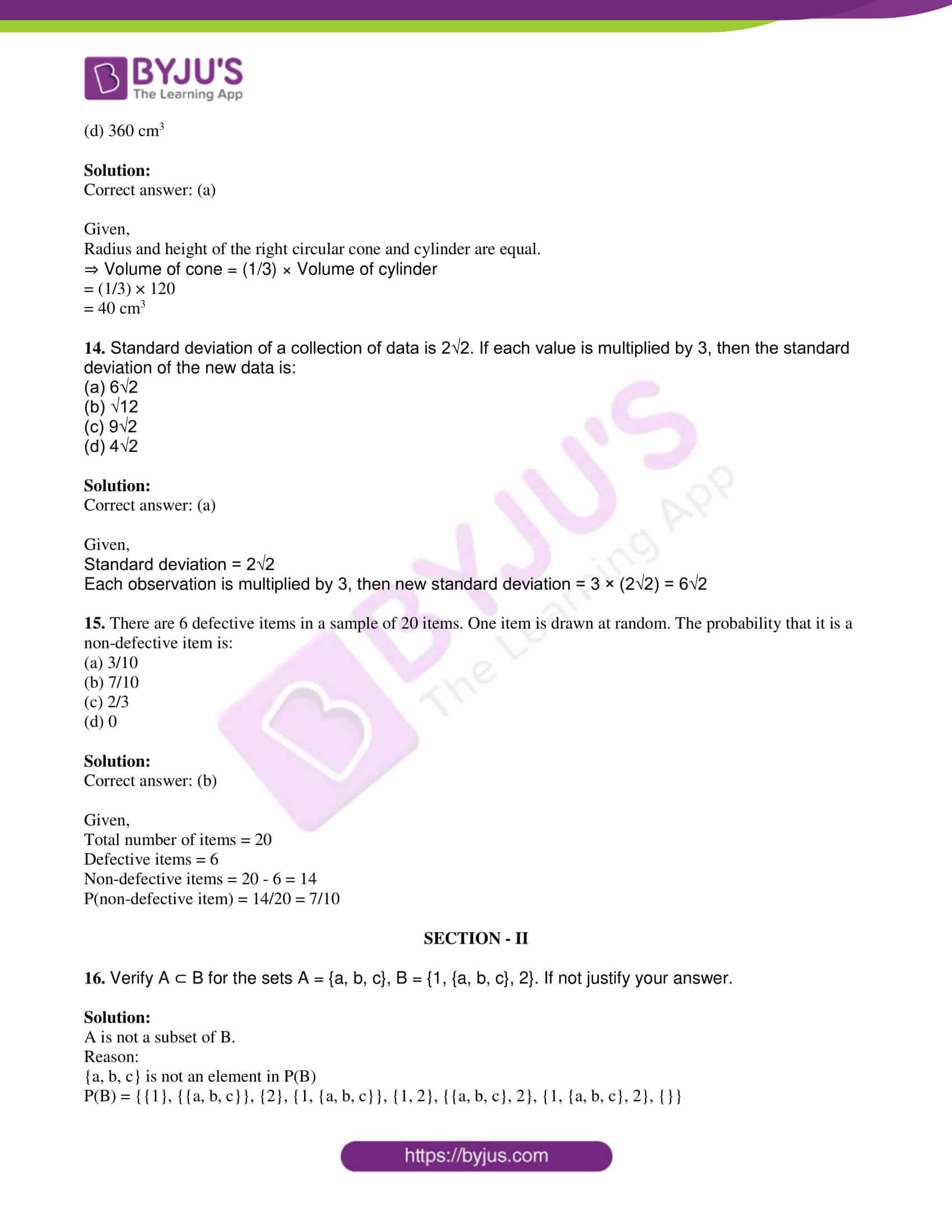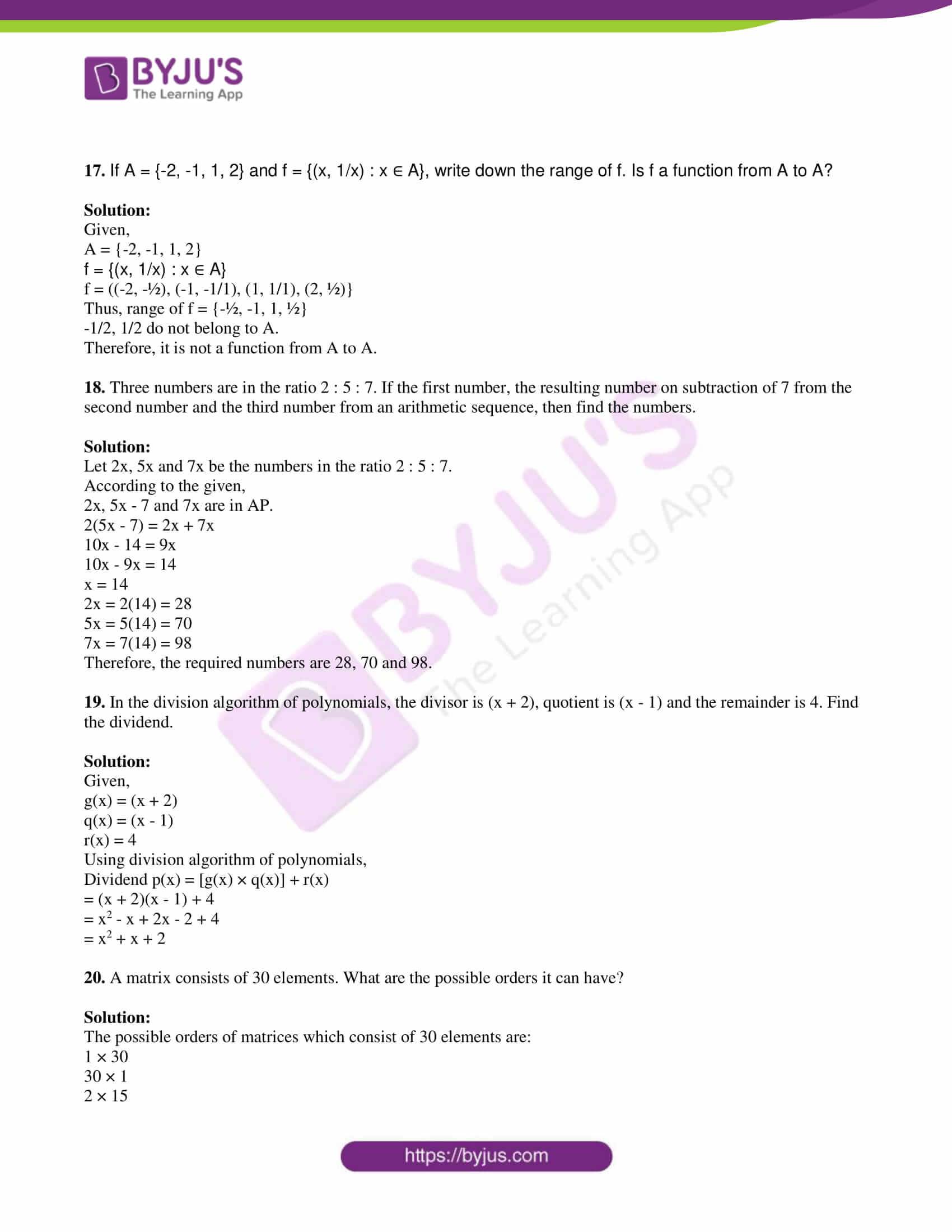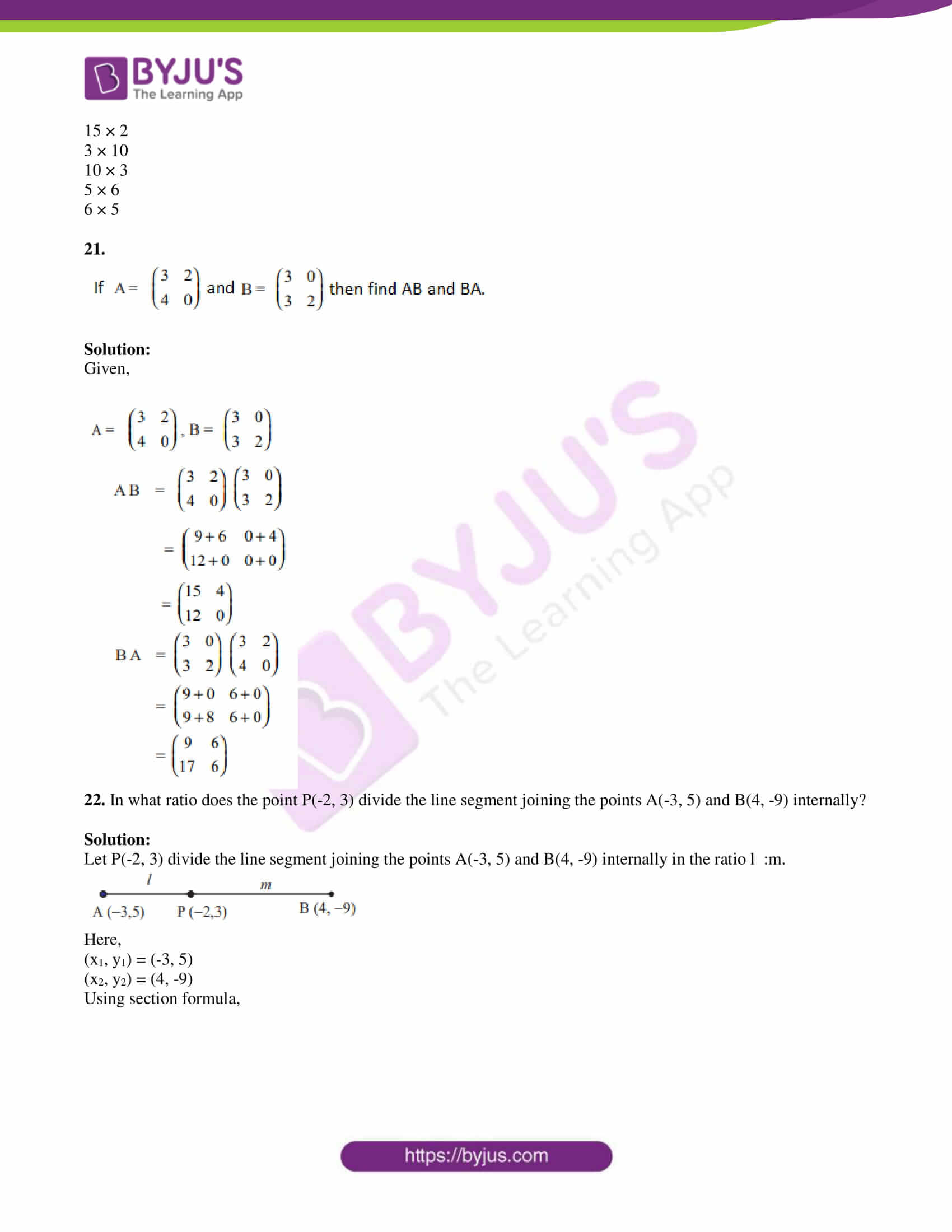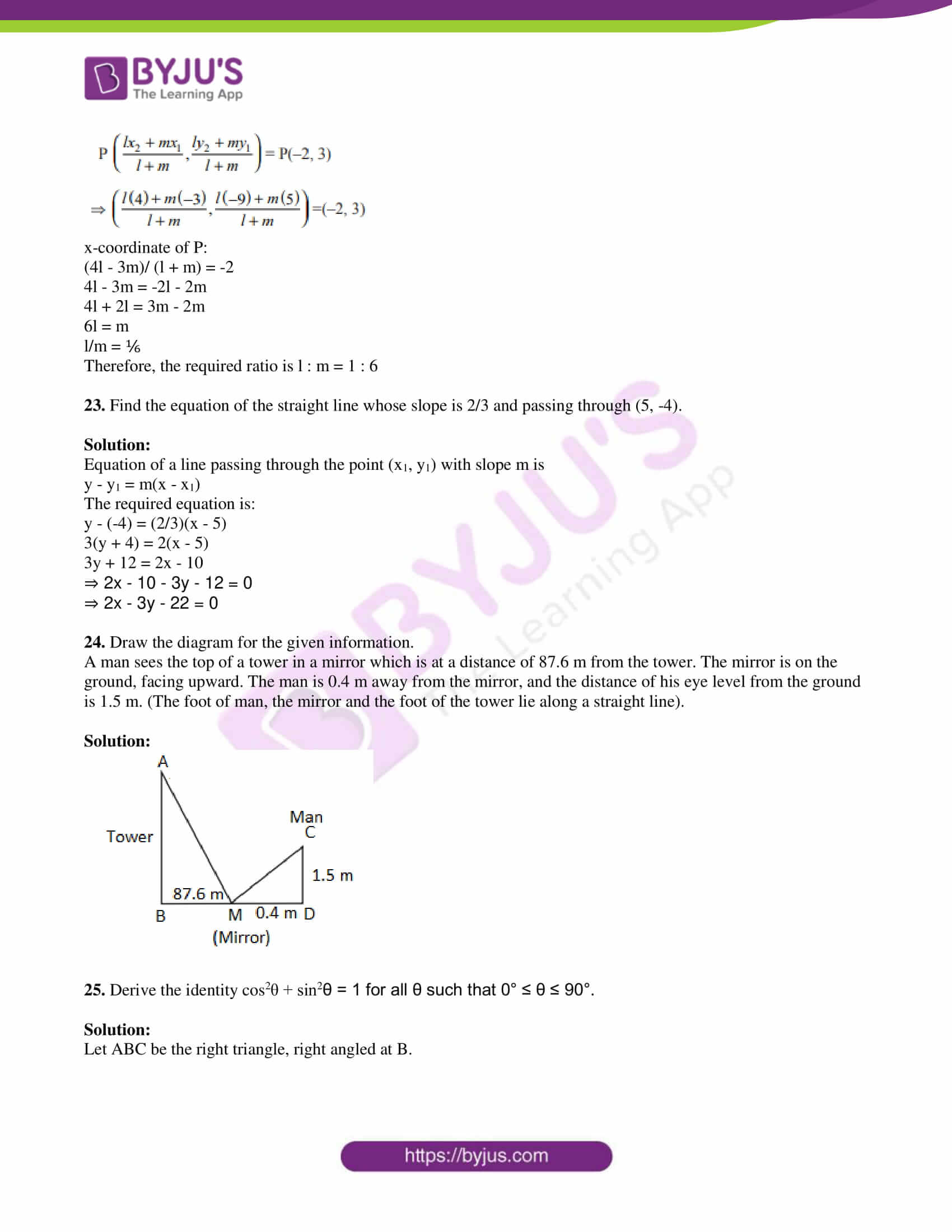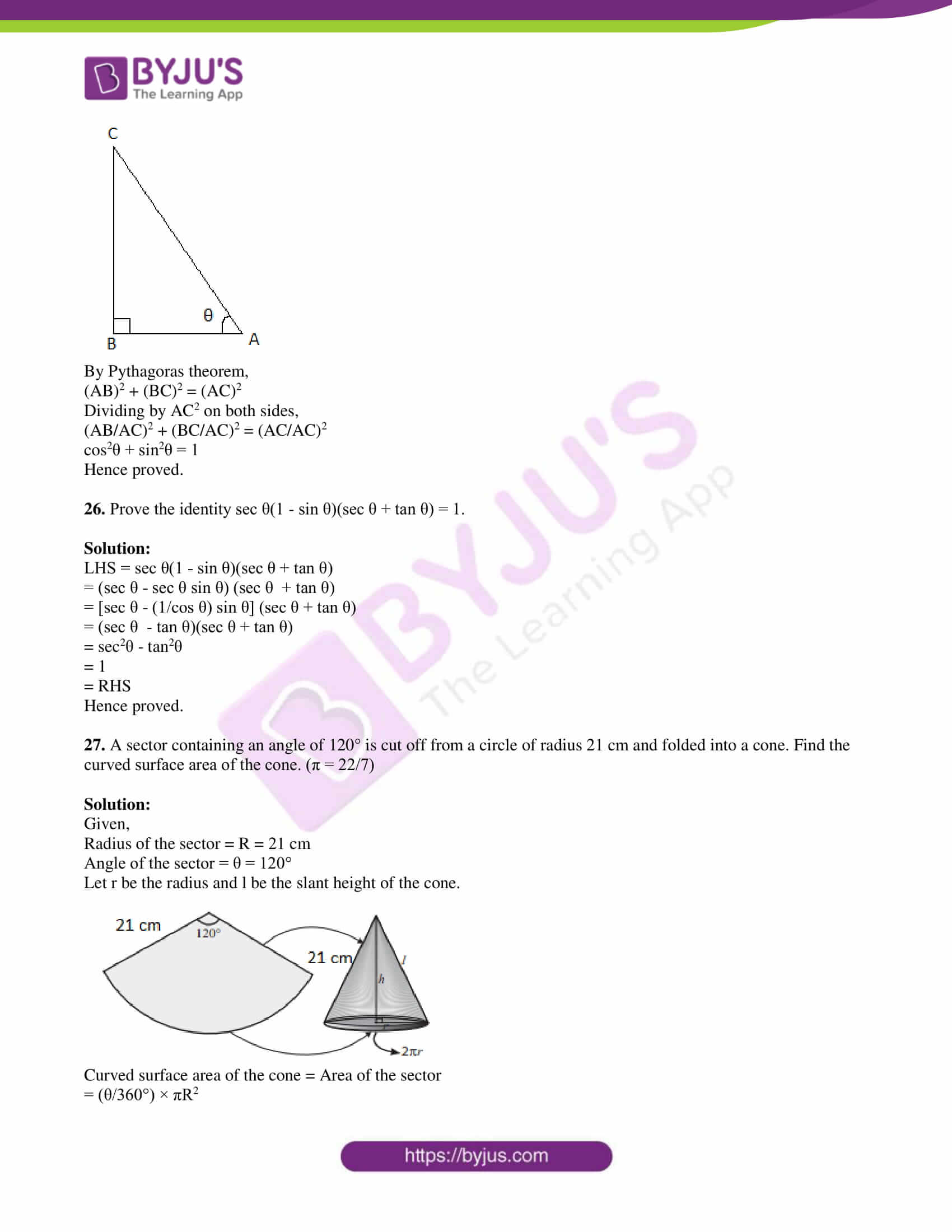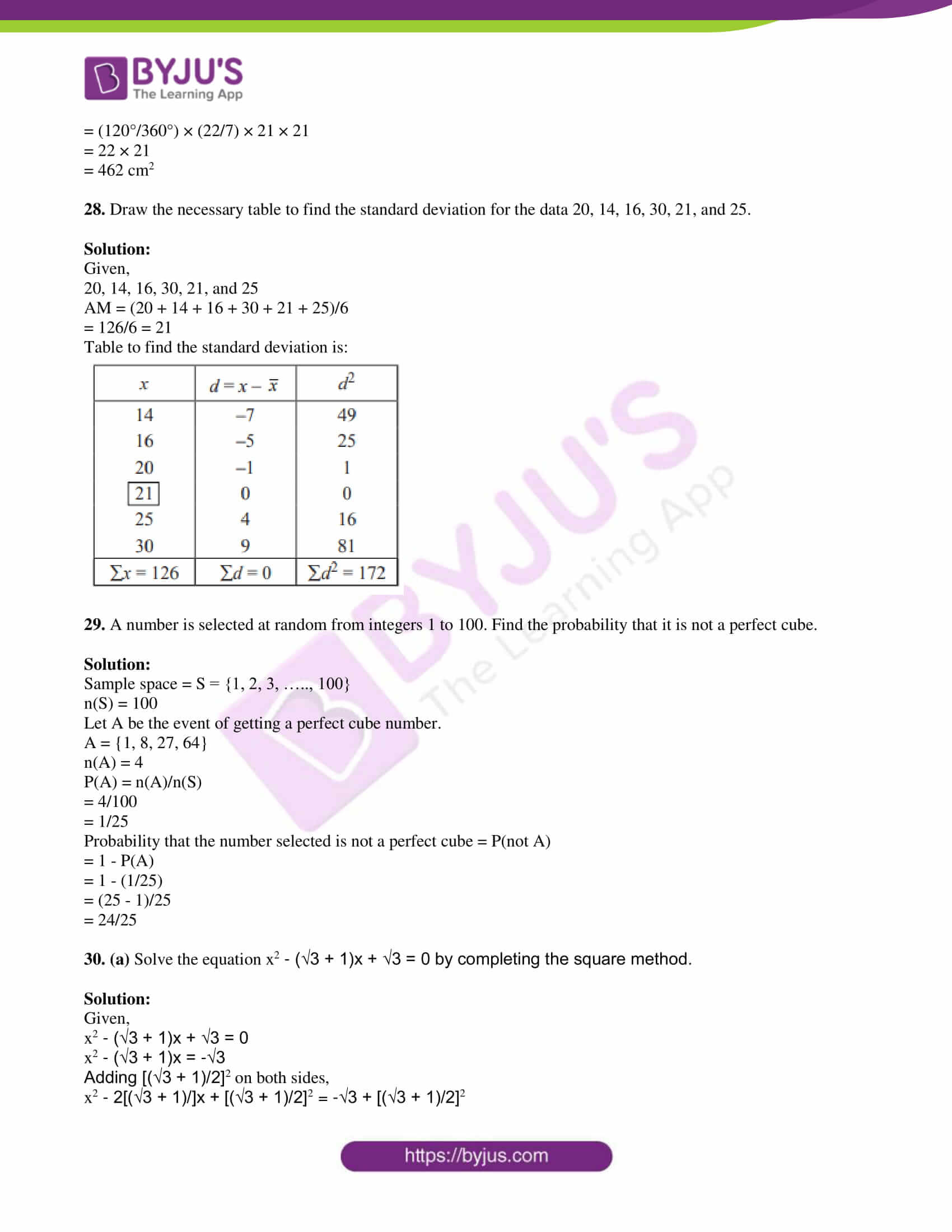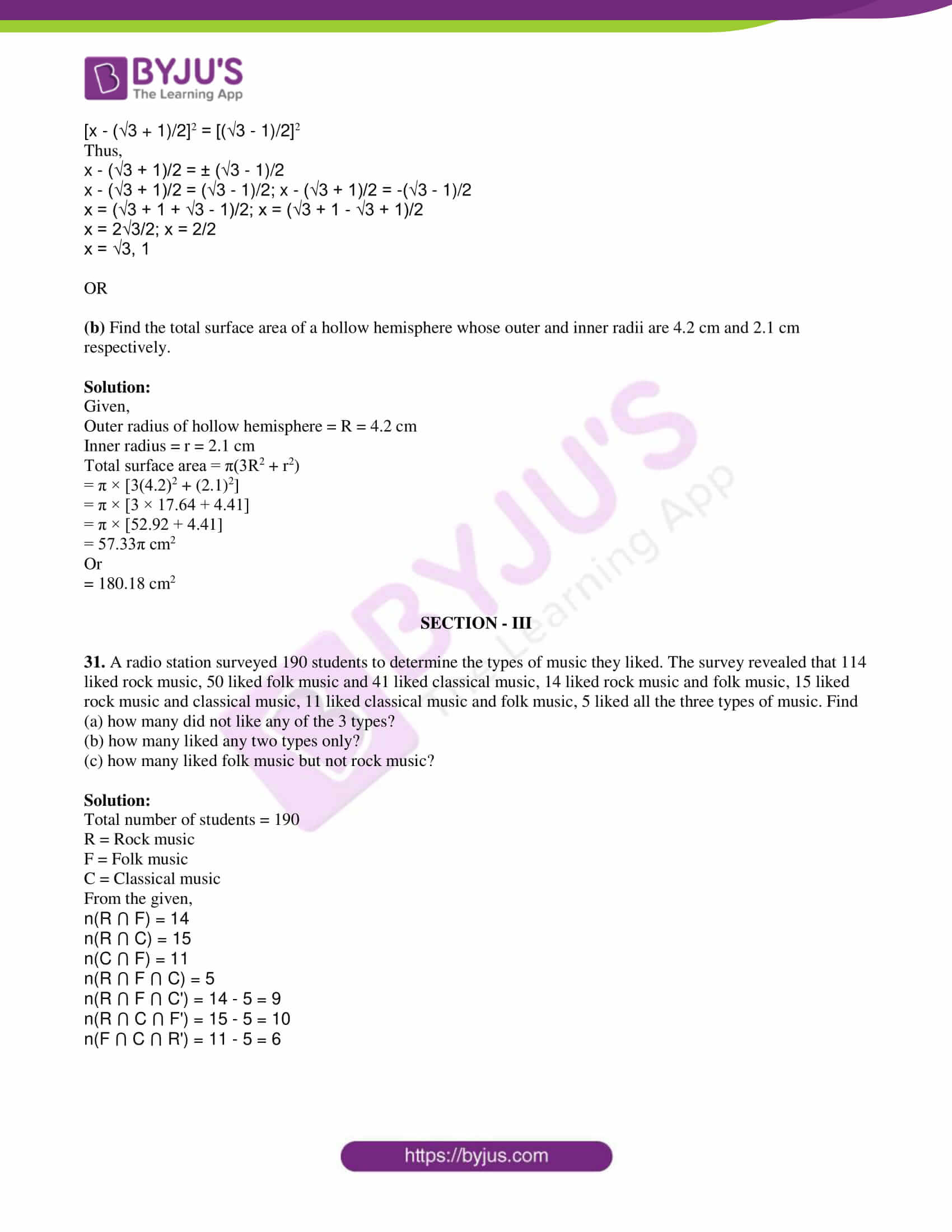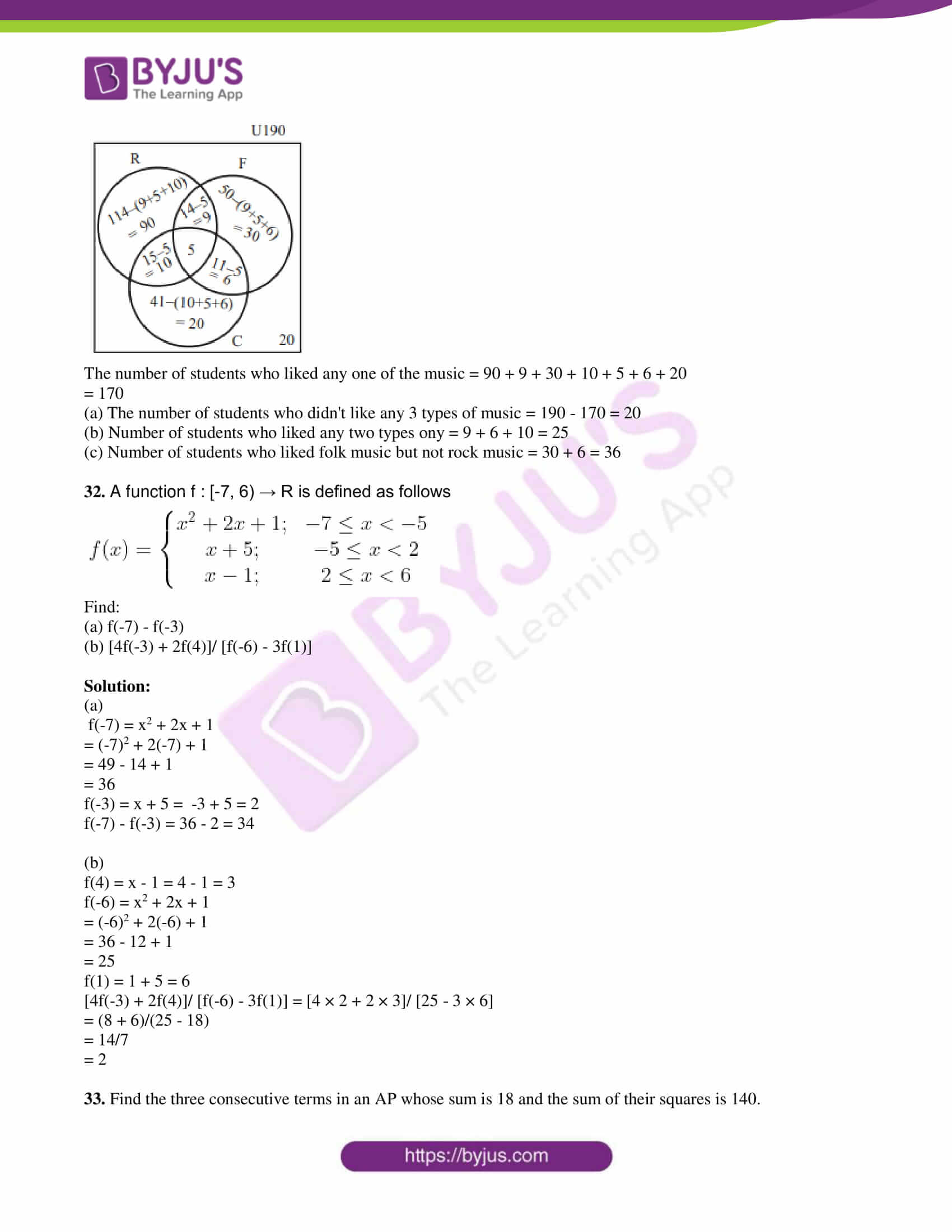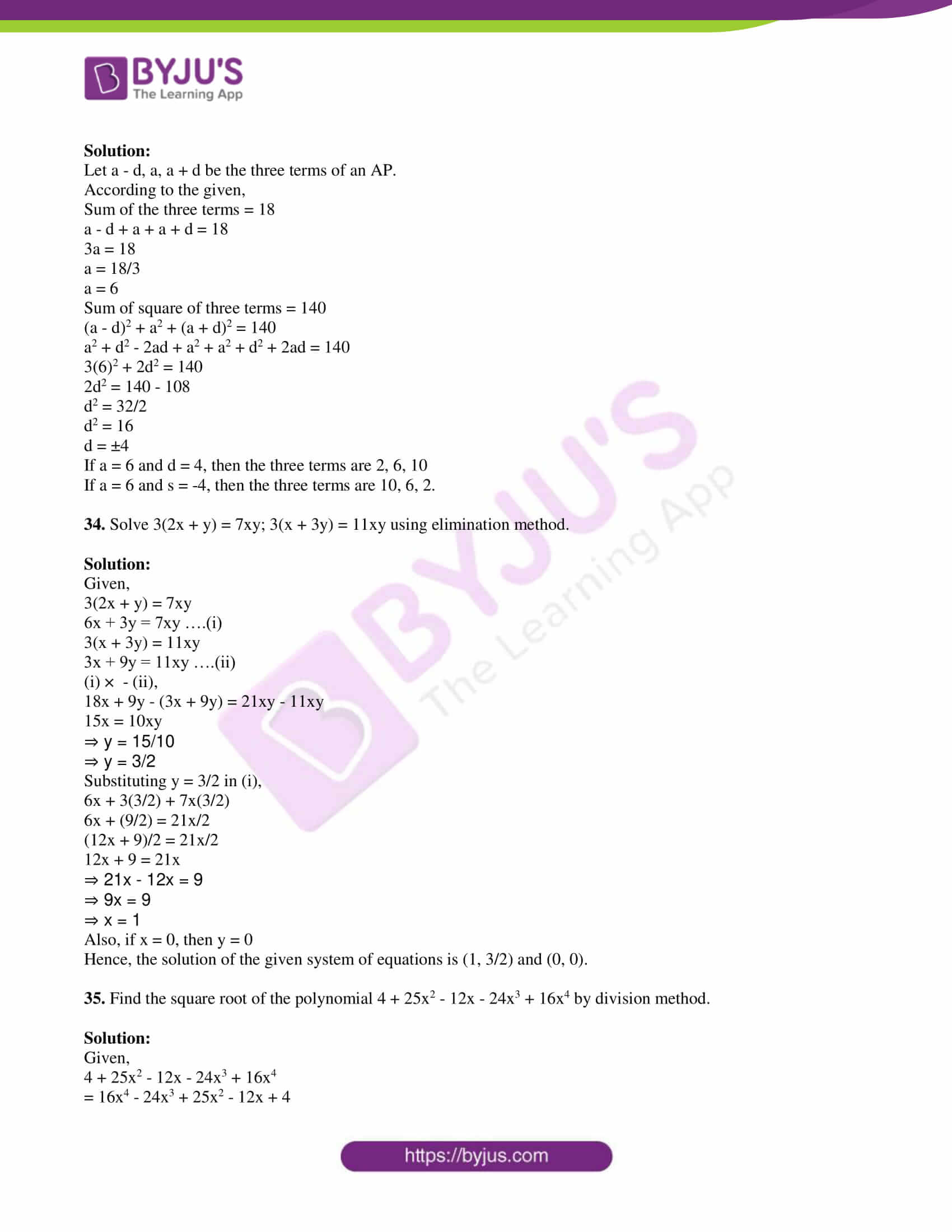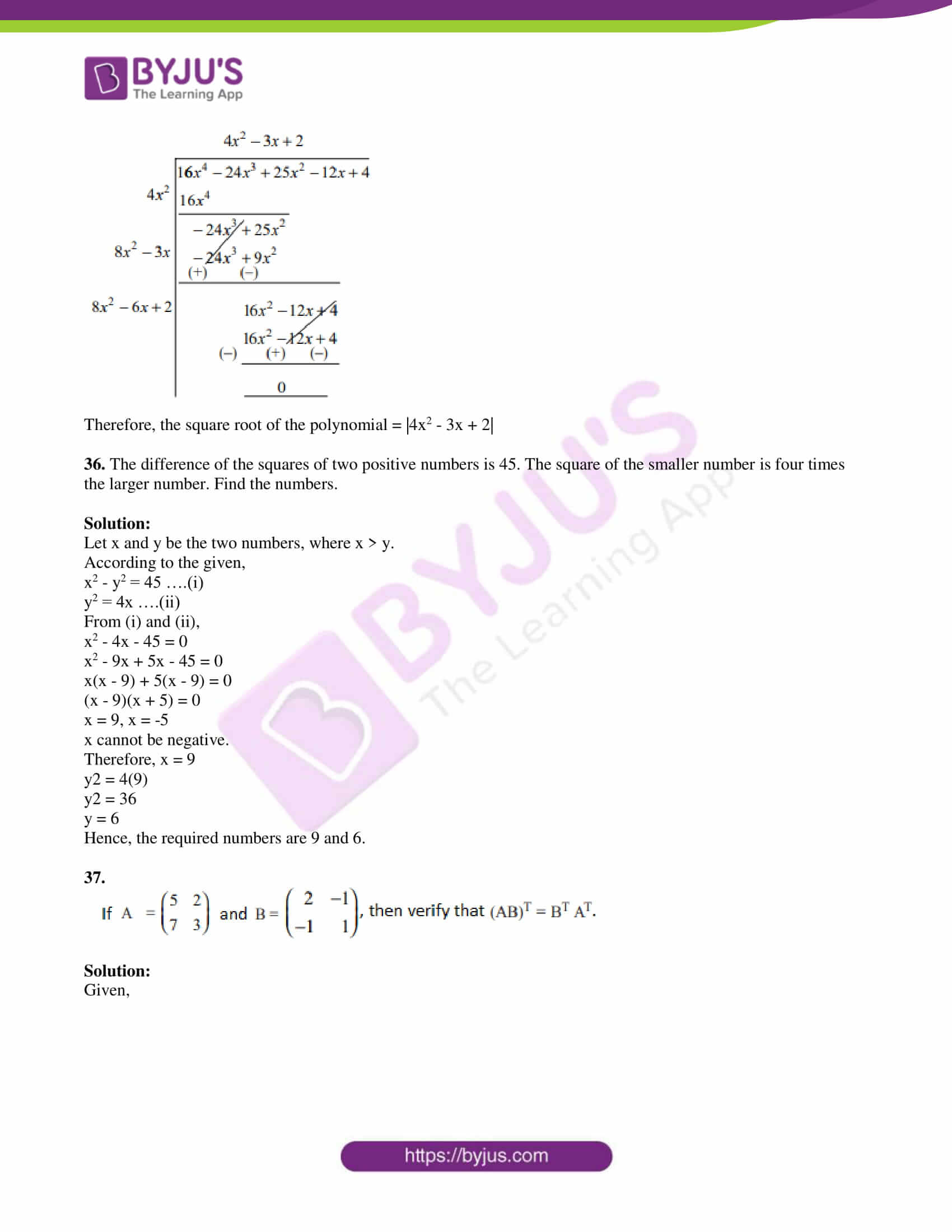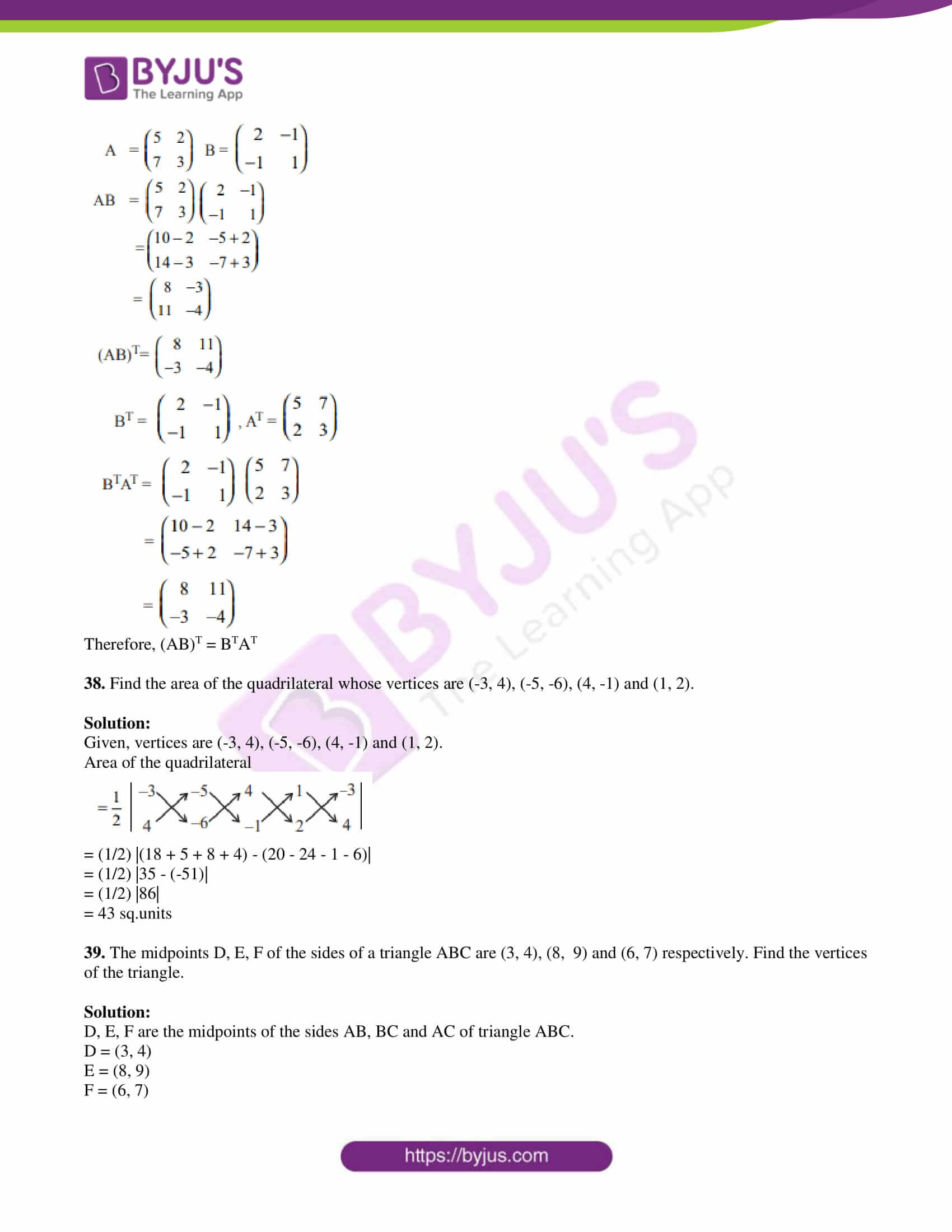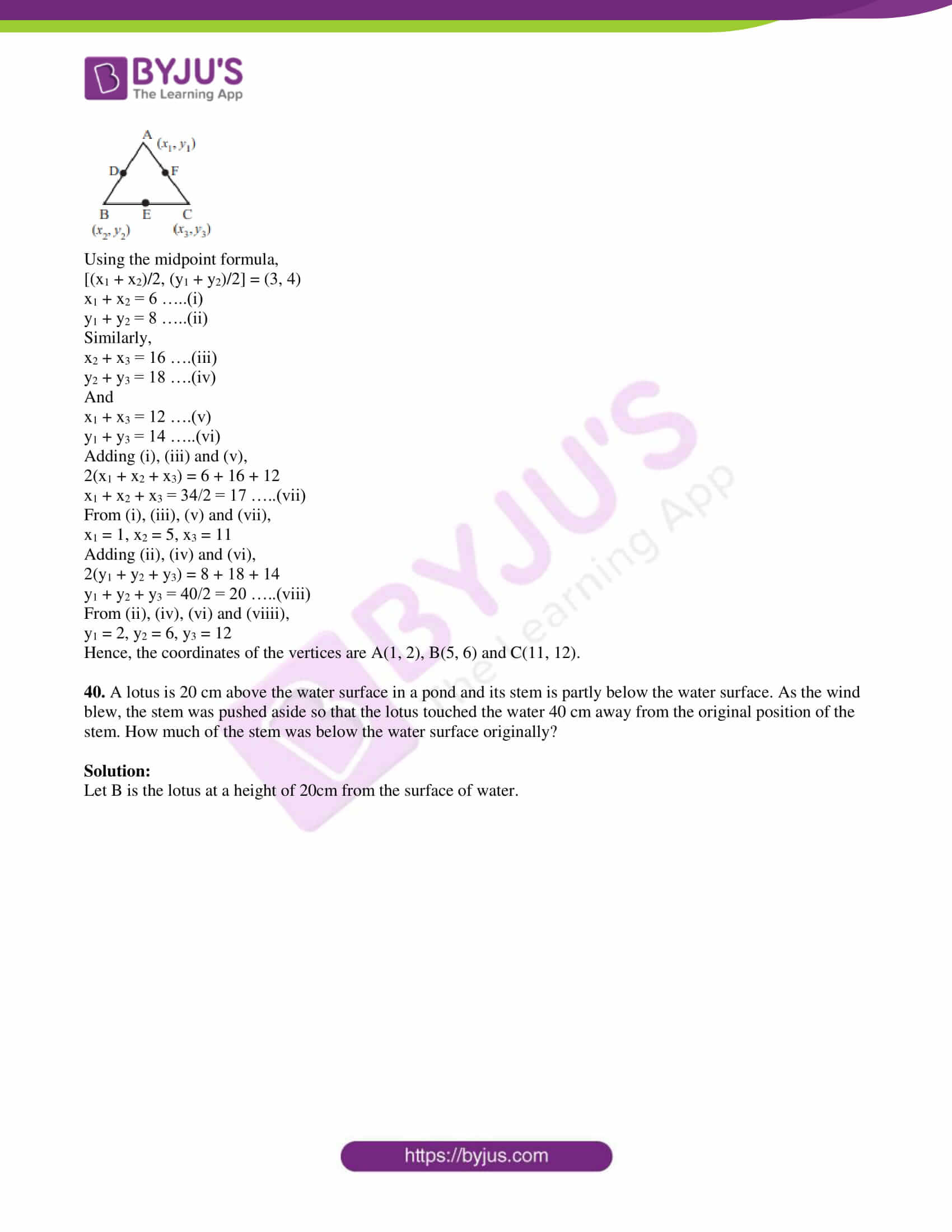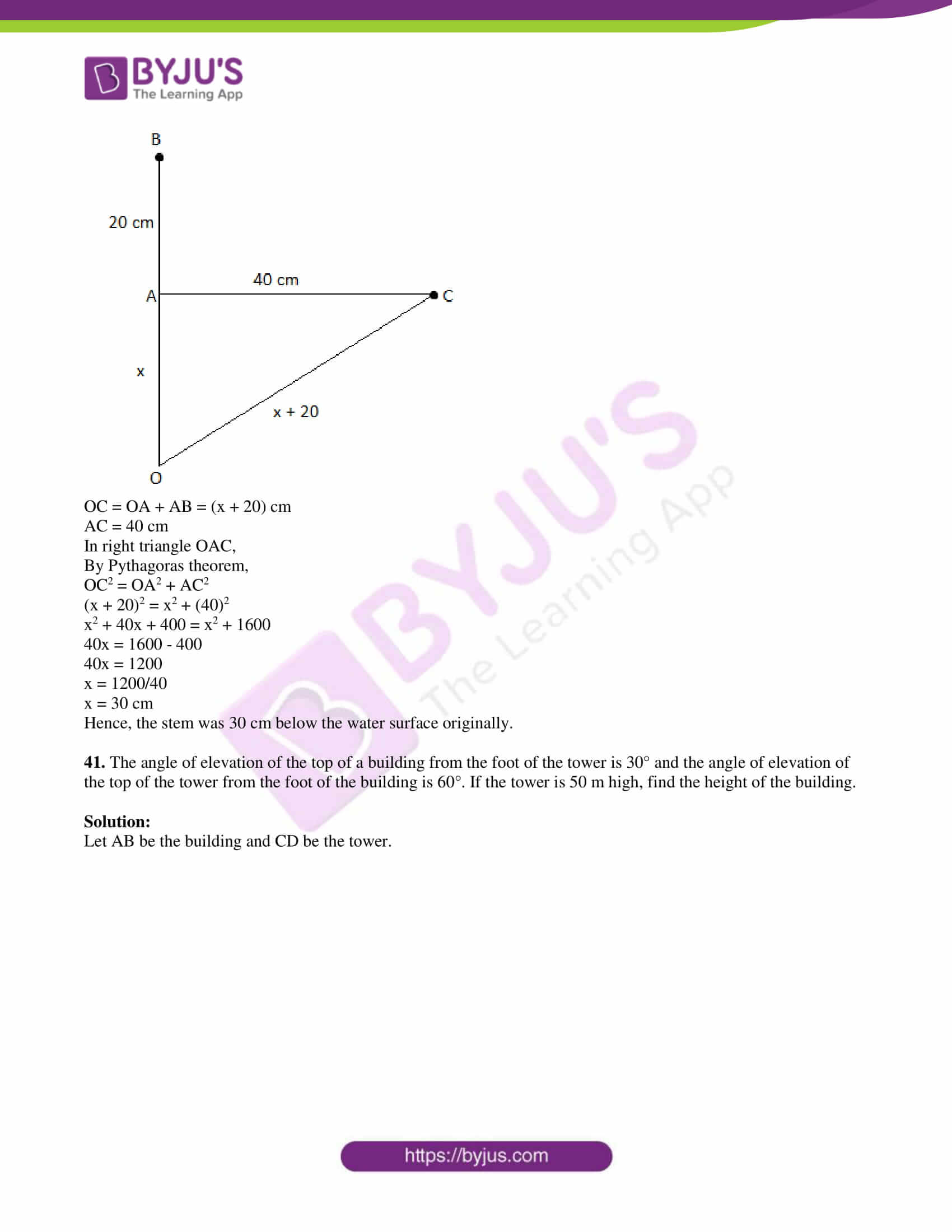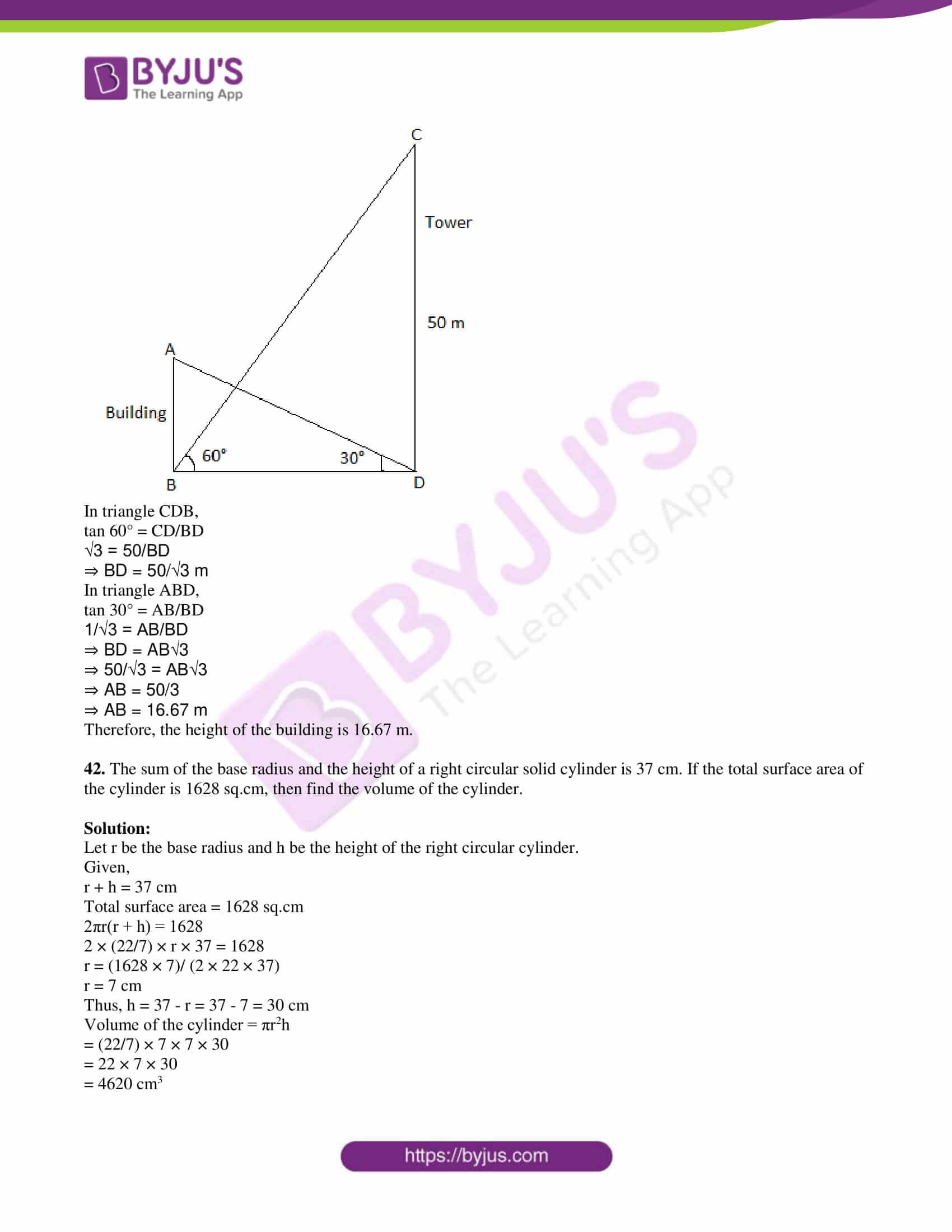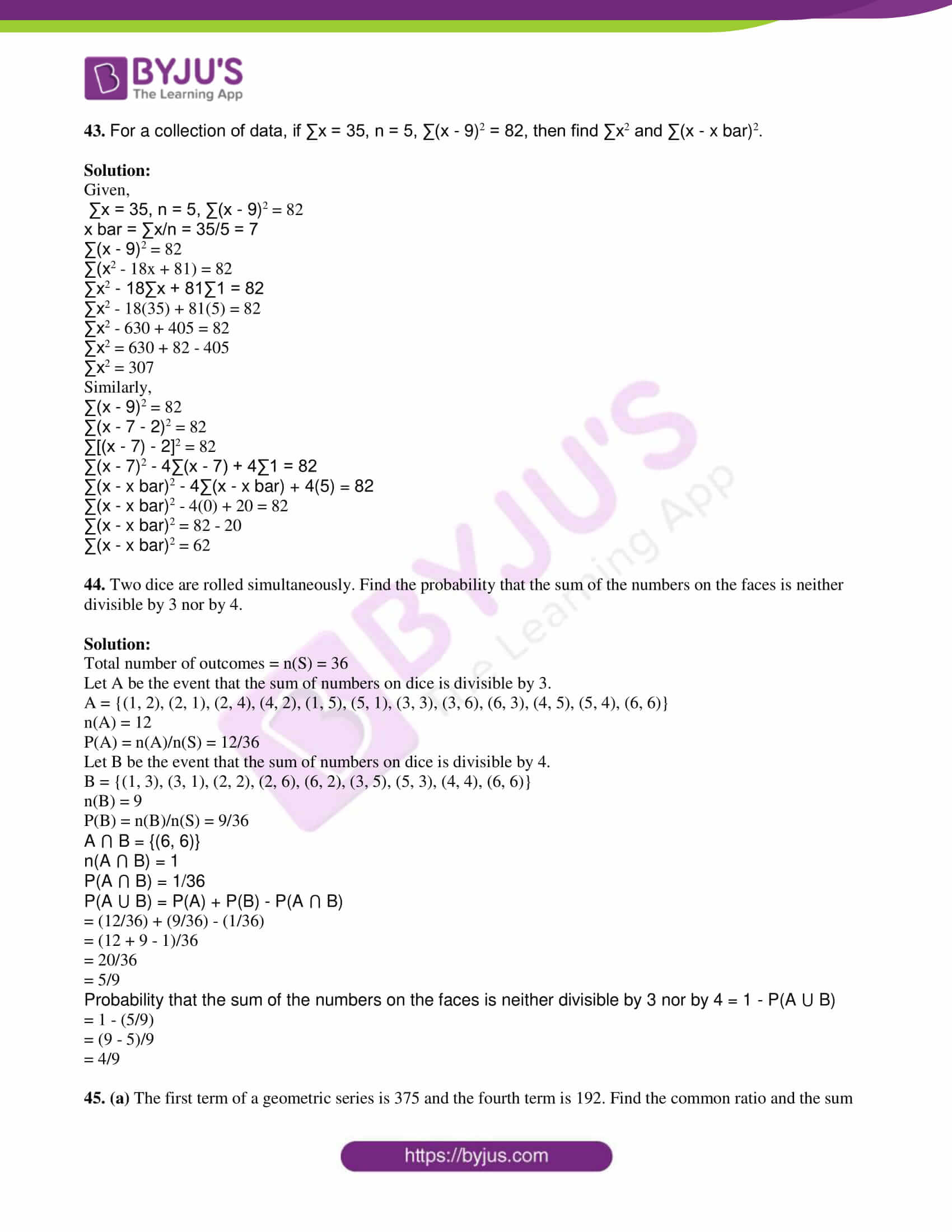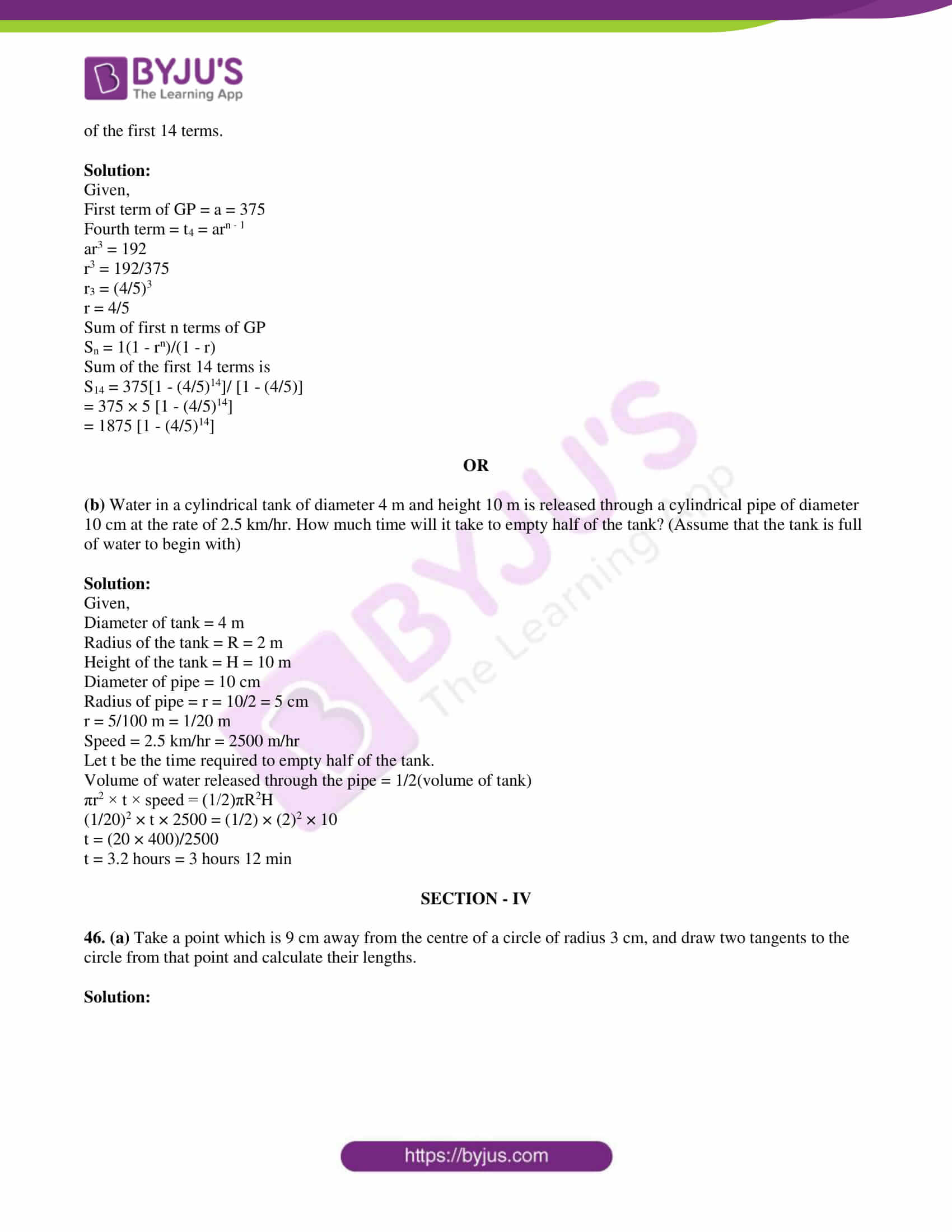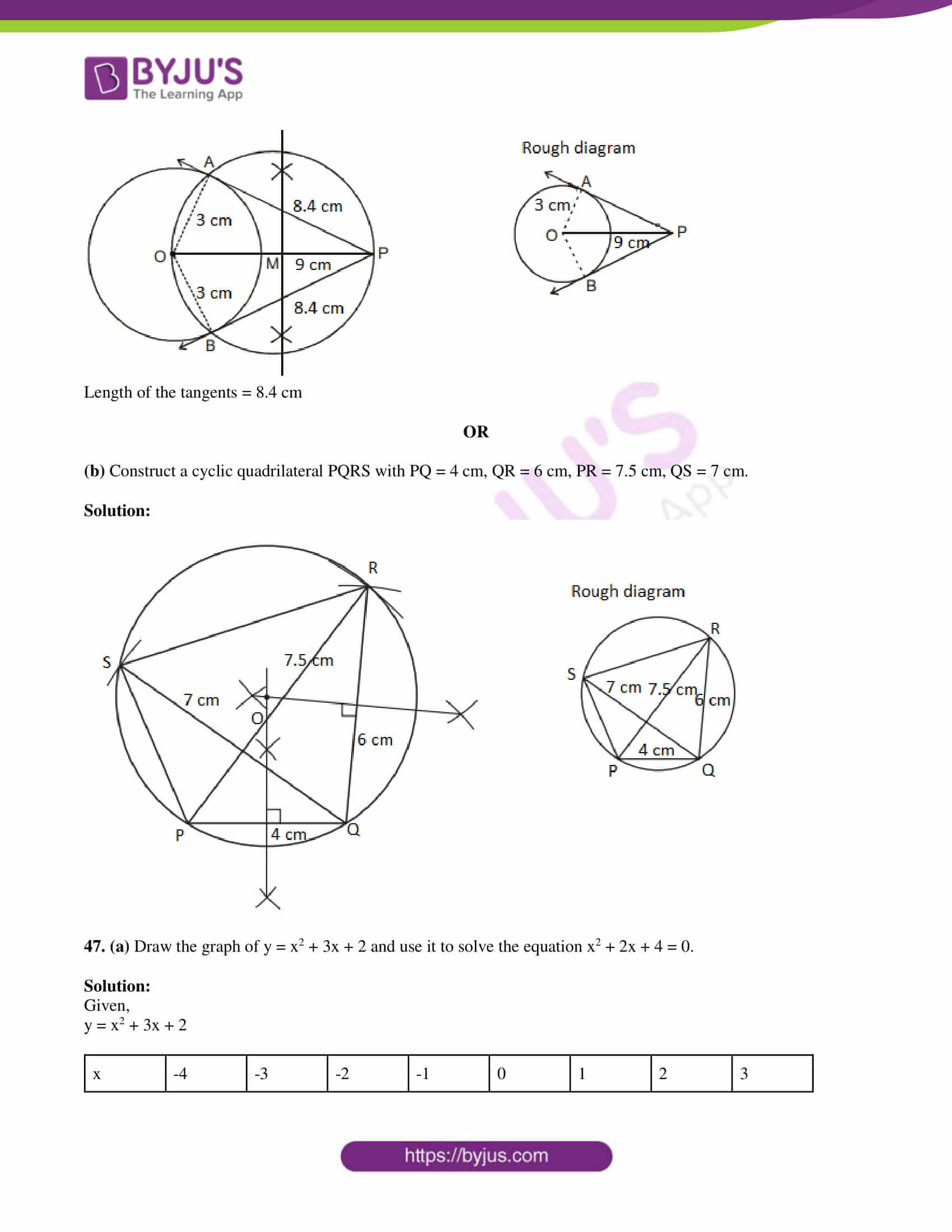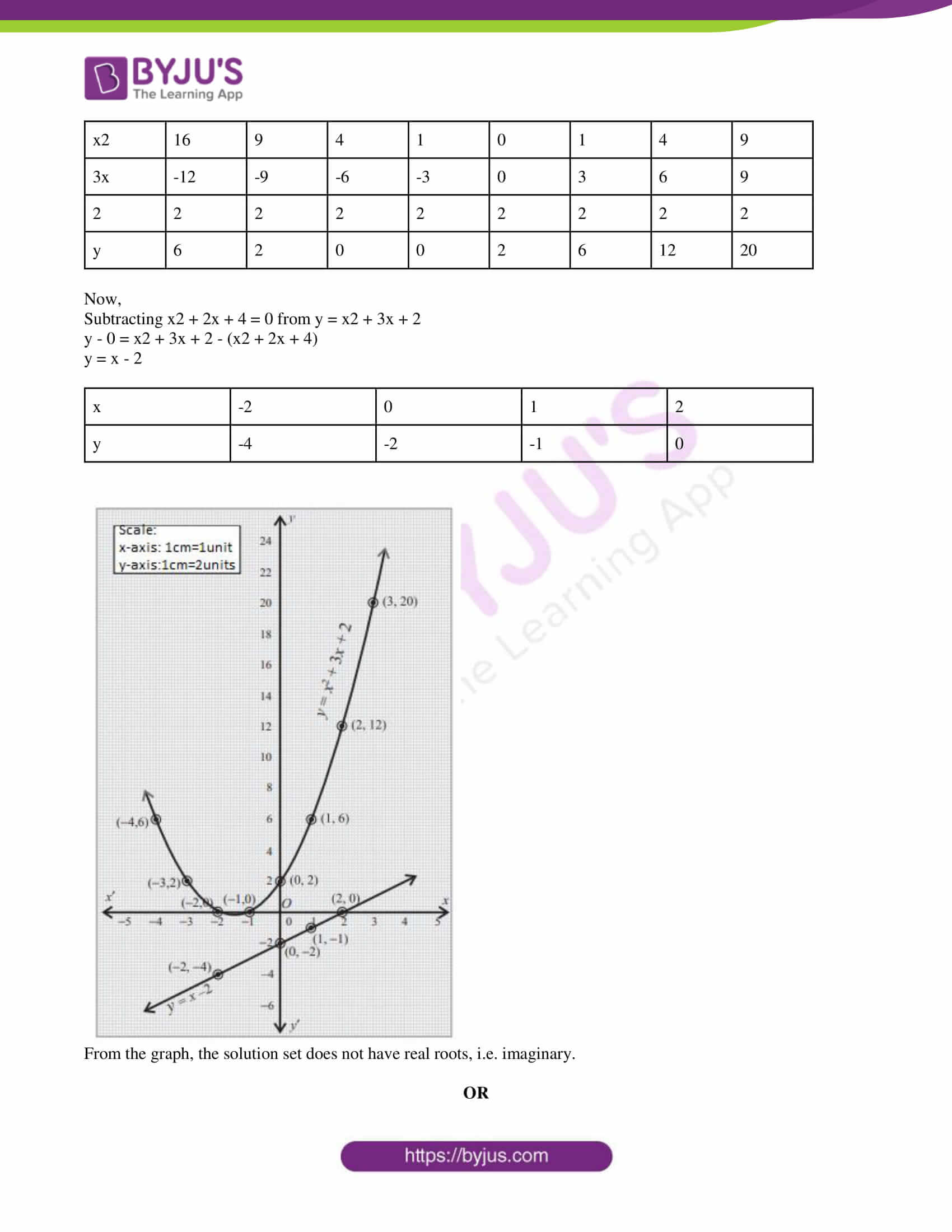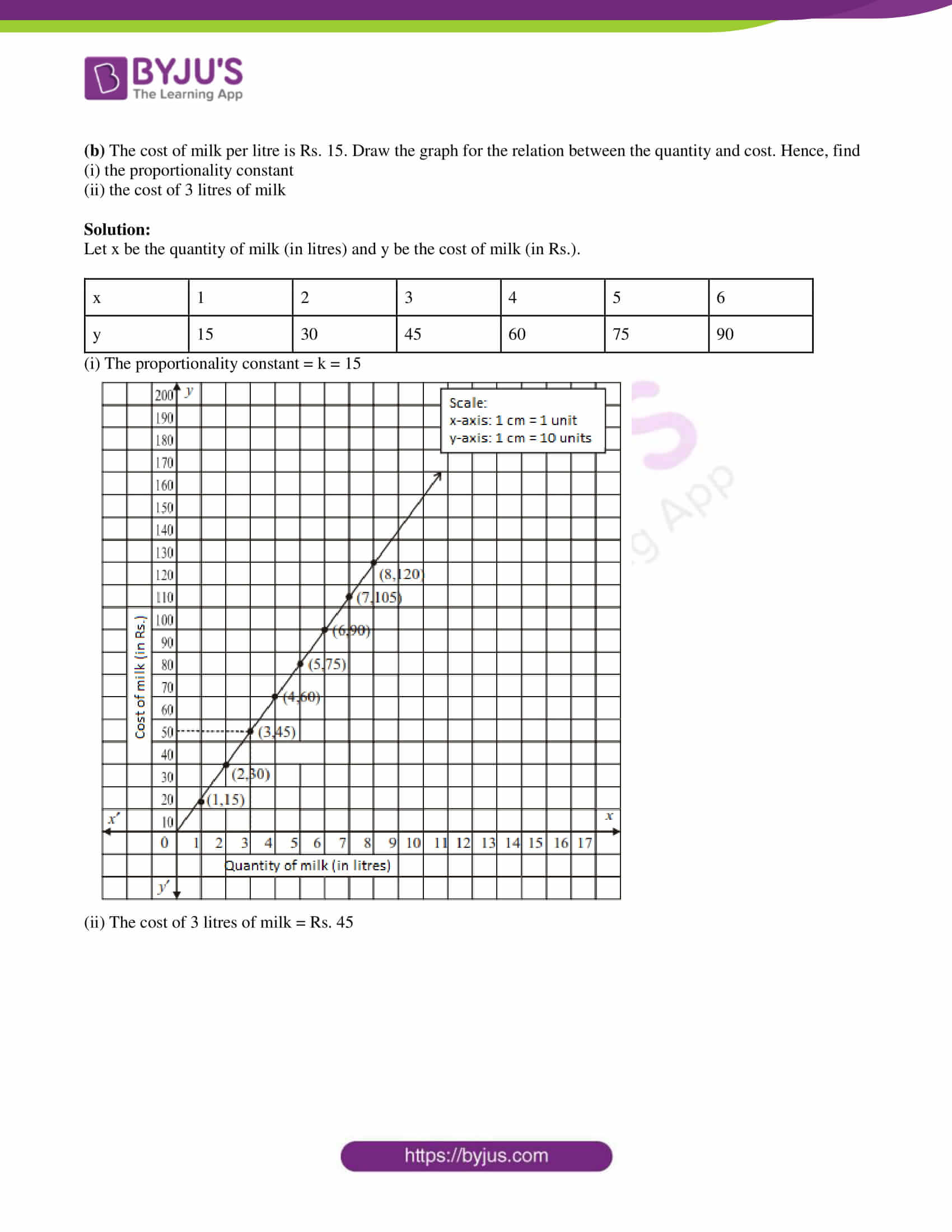SECTION – I

1. An example for a function which is not a relation, (Domain – R, Co-domain – R) is:

(a) y = x

(b) y = x – 1

(c) y = x2

(d) not possible

Solution:

We know that every function is a relation but it is not necessary that every relation be a function.

Therefore, y = x, y = x – 1 and y = x2 are functions.

2. If a, b, c are in GP, then (a – b)/(b – c) is equal to:

(a) a/c

(b) a/b

(c) c/b

(d) b/a

Solution:

Given,

a, b, c are in GP.

b2 = ac

Now,

(a – b)/(b – c)

Multiply and divide by b,

(ab – b2)/ (b2 – bc)

= (ab – ac)/ (b2 – bc)

= [a(b – c)]/ [b(b – c)]

= a/b

3. The next term of the series √3, √12, √27,… is:

(a) √39

(b) √32

(c) √54

(d) √48

Solution:

Given,

√3,√12,√27,….

a = √3

d = √12 – √3

= 2√3 – √3

= √3

Next term of the given series is:

√27 + √3

= 3√3 + √3

= 4√3

= √48

4. What can be the degree of the remainder atmost, when a fourth degree polynomial is divided by a quadratic polynomial?

(a) 2

(b) 0

(c) 4

(d) 1

Solution:

We know that any polynomial of degree four is divided by the quadratic polynomial, then the degree of the remainder must be less than the degree of the quadratic polynomial.

Therefore, maximum possible degree of remainder = 1

5. The LCM of x3 – a3 and (x – a)2 is:

(a) (x – a)2(x2 + ax + a2)

(b) (x3 – a3)(x + a)

(c) (x + a)2(x2 + ax + a2)

(d) (x3 – a3)(x – a)2

Solution:

Given,

x3 – a3 and (x – a)2

(x3 – a3) = (x – a)(x2 + ax + a2)

Hence, the LCM is (x – a)2(x2 + ax + a2)

6.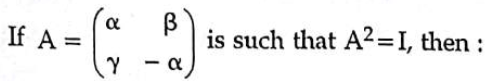(a) 1 – α2 – βγ = 0

(b) 1 + α2 + βγ = 0

(c) 1 + α2 – βγ = 0

(d) 1 – α2 + βγ = 0

Solution:

Given,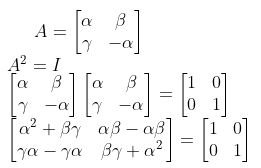Thus, α2 + βγ = 1

⇒ 1 – α2 – βγ = 0

7. Slope of the straight line which is perpendicular to the straight line joining the points (-2, 6) and (4, 8) is equal to:

(a) -3

(b) 1/3

(c) -1/3

(d) 3

Solution:

Let the given points be:

(x1, y1) = (-2, 6)

(x2, y2) = (4, 8)

Slope of the line joining the given points = m = (y2 – y1)/(x2 – x1)

= (8 – 6)/(4 + 2)

= 2/6

= ⅓

Slope of the straight line which is perpendicular to the straight line joining the given points = -1/m

= -1/(⅓)

= -3

8. The centre of a circle is (-6, 4). If one end of the diameter of the circle is at (-12, 8), then the other end is at:

(a) (-3, 2)

(b) (-18, 12)

(c) (0, 0)

(d) (-9, 6)

Solution:

Given,

Centre = (-6, 4)

One end of the diameter = (-12, 8)

Let (x, y) be the other end of the diameter.

We know that, centre is the midpoint of diameter.

(-6, 4) = [(-12 + x)/2, (8 + y)/2]

-12 + x = -12; 8 + y = 8

x = 12 – 12; y = 8 – 8

x =0, y = 0

Hence, the required point is (0, 0).

9. The area of two similar triangles are 16 cm2 and 36 cm2 respectively. If the altitude of the first triangle is 3 cm, then the corresponding altitude of the other triangle is:

(a) 4 cm

(b) 6.5 cm

(c) 4.5 cm

(d) 6 cm

Solution:

Let x be the altitude of the second triangle.

We know that,

Area of first triangle/Area of second triangle = Square the altitude of first triangle/ Square the altitude of second triangle

16/36 = (3)2/x2

⇒ x2 = (9 × 36)/16

⇒ x2 = (9/2)2

⇒ x = 9/2

⇒ x = 4.5 cm

10. If a vertical stick of 12 m long casts a shadow of 8 m long on the ground and at the same time, a tower casts a shadow of 40 m long on the ground, then the height of the tower is:

(a) 75 m

(b) 40 m

(c) 60 m

(d) 50 m

Solution:

Given,

Height of the stick = 12 m

Length of the shadow of stick = 8 m

Let x be the height of the tower.

Length of the shadow of tower = 40 m

Thus,

12/8 = x/40

⇒ x = (12 × 40)/8

⇒ x = 60 m

11. (1 + cot2θ)(1 – cos θ)(1 + cos θ) =

(a) sec2θ – tan2θ

(b) tan2θ – sec2θ

(c) cos2θ – sin2θ

(d) sin2θ – cos2θ

Solution:

(1 + cot2θ)(1 – cos θ)(1 + cos θ)

= cosec2θ(1 – cos2θ)

= cosec2θ sin2θ

= (1/sin2θ) sin2θ

= 1

= sec2θ – tan2θ

12. If A is an acute angle of a ΔABC, right angles at B, then the value of sin A + cos A is:

(a) less than one

(b) equal to one

(c) equal to two

(d) greater than one

Solution:

Given,

A is an acute angle of a ΔABC, right angles at B.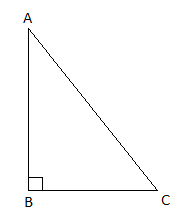sin A + cos A = (BC/AC) + (AB + AC)

= (BC + AB)/AC

We know that the sum of two sides of a triangle is greater than the third side.

Here, BC + AB > AC

Thus, sin A + cos A > 1

13. Radius and height of a right circular cone and that of a right circular cylinder are respectively equal. If the volume of the cylinder is 120 cm3, then the volume of the cone is equal to:

(a) 40 cm3

(b) 1200 cm3

(c) 90 cm3

(d) 360 cm3

Solution:

Given,

Radius and height of the right circular cone and cylinder are equal.

⇒ Volume of cone = (1/3) × Volume of cylinder

= (1/3) × 120

= 40 cm3

14. Standard deviation of a collection of data is 2√2. If each value is multiplied by 3, then the standard deviation of the new data is:

(a) 6√2

(b) √12

(c) 9√2

(d) 4√2

Solution:

Given,

Standard deviation = 2√2

Each observation is multiplied by 3, then new standard deviation = 3 × (2√2) = 6√2

15. There are 6 defective items in a sample of 20 items. One item is drawn at random. The probability that it is a non-defective item is:

(a) 3/10

(b) 7/10

(c) 2/3

(d) 0

Solution:

Given,

Total number of items = 20

Defective items = 6

Non-defective items = 20 – 6 = 14

P(non-defective item) = 14/20 = 7/10

SECTION – II

16. Verify A ⊂ B for the sets A = {a, b, c}, B = {1, {a, b, c}, 2}. If not justify your answer.

Solution:

A is not a subset of B.

Reason:

{a, b, c} is not an element in P(B)

P(B) = {{1}, {{a, b, c}}, {2}, {1, {a, b, c}}, {1, 2}, {{a, b, c}, 2}, {1, {a, b, c}, 2}, {}}

17. If A = {-2, -1, 1, 2} and f = {(x, 1/x) : x ∈ A}, write down the range of f. Is f a function from A to A?

Solution:

Given,

A = {-2, -1, 1, 2}

f = {(x, 1/x) : x ∈ A}

f = ((-2, -½), (-1, -1/1), (1, 1/1), (2, ½)}

Thus, range of f = {-½, -1, 1, ½}

-1/2, 1/2 do not belong to A.

Therefore, it is not a function from A to A.

18. Three numbers are in the ratio 2 : 5 : 7. If the first number, the resulting number on subtraction of 7 from the second number and the third number from an arithmetic sequence, then find the numbers.

Solution:

Let 2x, 5x and 7x be the numbers in the ratio 2 : 5 : 7.

According to the given,

2x, 5x – 7 and 7x are in AP.

2(5x – 7) = 2x + 7x

10x – 14 = 9x

10x – 9x = 14

x = 14

2x = 2(14) = 28

5x = 5(14) = 70

7x = 7(14) = 98

Therefore, the required numbers are 28, 70 and 98.

19. In the division algorithm of polynomials, the divisor is (x + 2), quotient is (x – 1) and the remainder is 4. Find the dividend.

Solution:

Given,

g(x) = (x + 2)

q(x) = (x – 1)

r(x) = 4

Using division algorithm of polynomials,

Dividend p(x) = [g(x) × q(x)] + r(x)

= (x + 2)(x – 1) + 4

= x2 – x + 2x – 2 + 4

= x2 + x + 2

20. A matrix consists of 30 elements. What are the possible orders it can have?

Solution:

The possible orders of matrices which consist of 30 elements are:

1 × 30

30 × 1

2 × 15

15 × 2

3 × 10

10 × 3

5 × 6

6 × 5

21.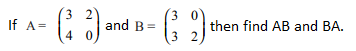Solution:

Given,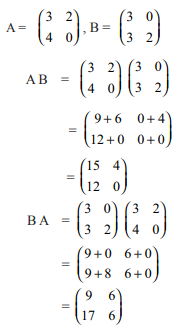22. In what ratio does the point P(-2, 3) divide the line segment joining the points A(-3, 5) and B(4, -9) internally?

Solution:

Let P(-2, 3) divide the line segment joining the points A(-3, 5) and B(4, -9) internally in the ratio l :m.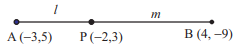Here,

(x1, y1) = (-3, 5)

(x2, y2) = (4, -9)

Using section formula,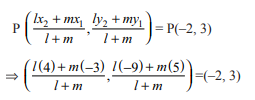x-coordinate of P:

(4l – 3m)/ (l + m) = -2

4l – 3m = -2l – 2m

4l + 2l = 3m – 2m

6l = m

l/m = ⅙

Therefore, the required ratio is l : m = 1 : 6

23. Find the equation of the straight line whose slope is 2/3 and passing through (5, -4).

Solution:

Equation of a line passing through the point (x1, y1) with slope m is

y – y1 = m(x – x1)

The required equation is:

y – (-4) = (2/3)(x – 5)

3(y + 4) = 2(x – 5)

3y + 12 = 2x – 10

⇒ 2x – 10 – 3y – 12 = 0

⇒ 2x – 3y – 22 = 0

24. Draw the diagram for the given information.

A man sees the top of a tower in a mirror which is at a distance of 87.6 m from the tower. The mirror is on the ground, facing upward. The man is 0.4 m away from the mirror, and the distance of his eye level from the ground is 1.5 m. (The foot of man, the mirror and the foot of the tower lie along a straight line).

Solution: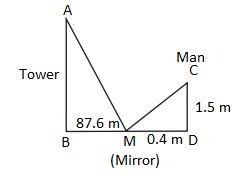25. Derive the identity cos2θ + sin2θ = 1 for all θ such that 0° ≤ θ ≤ 90°.

Solution:

Let ABC be the right triangle, right angled at B.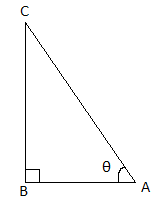By Pythagoras theorem,

(AB)2 + (BC)2 = (AC)2

Dividing by AC2 on both sides,

(AB/AC)2 + (BC/AC)2 = (AC/AC)2

cos2θ + sin2θ = 1

Hence proved.

26. Prove the identity sec θ(1 – sin θ)(sec θ + tan θ) = 1.

Solution:

LHS = sec θ(1 – sin θ)(sec θ + tan θ)

= (sec θ – sec θ sin θ) (sec θ + tan θ)

= [sec θ – (1/cos θ) sin θ] (sec θ + tan θ)

= (sec θ – tan θ)(sec θ + tan θ)

= sec2θ – tan2θ

= 1

= RHS

Hence proved.

27. A sector containing an angle of 120° is cut off from a circle of radius 21 cm and folded into a cone. Find the curved surface area of the cone. (π = 22/7)

Solution:

Given,

Radius of the sector = R = 21 cm

Angle of the sector = θ = 120°

Let r be the radius and l be the slant height of the cone.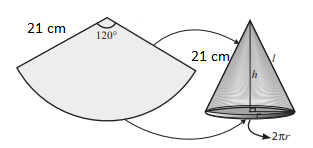Curved surface area of the cone = Area of the sector

= (θ/360°) × πR2

= (120°/360°) × (22/7) × 21 × 21

= 22 × 21

= 462 cm2

28. Draw the necessary table to find the standard deviation for the data 20, 14, 16, 30, 21, and 25.

Solution:

Given,

20, 14, 16, 30, 21, and 25

AM = (20 + 14 + 16 + 30 + 21 + 25)/6

= 126/6 = 21

Table to find the standard deviation is: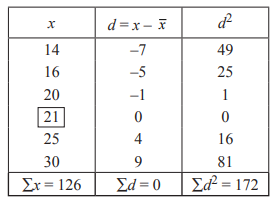29. A number is selected at random from integers 1 to 100. Find the probability that it is not a perfect cube.

Solution:

Sample space = S = {1, 2, 3, ….., 100}

n(S) = 100

Let A be the event of getting a perfect cube number.

A = {1, 8, 27, 64}

n(A) = 4

P(A) = n(A)/n(S)

= 4/100

= 1/25

Probability that the number selected is not a perfect cube = P(not A)

= 1 – P(A)

= 1 – (1/25)

= (25 – 1)/25

= 24/25

30. (a) Solve the equation x2 – (√3 + 1)x + √3 = 0 by completing the square method.

Solution:

Given,

x2 – (√3 + 1)x + √3 = 0

x2 – (√3 + 1)x = -√3

Adding [(√3 + 1)/2]2 on both sides,

x2 – 2[(√3 + 1)/]x + [(√3 + 1)/2]2 = -√3 + [(√3 + 1)/2]2

[x – (√3 + 1)/2]2 = [(√3 – 1)/2]2

Thus,

x – (√3 + 1)/2 = ± (√3 – 1)/2

x – (√3 + 1)/2 = (√3 – 1)/2; x – (√3 + 1)/2 = -(√3 – 1)/2

x = (√3 + 1 + √3 – 1)/2; x = (√3 + 1 – √3 + 1)/2

x = 2√3/2; x = 2/2

x = √3, 1

OR

(b) Find the total surface area of a hollow hemisphere whose outer and inner radii are 4.2 cm and 2.1 cm respectively.

Solution:

Given,

Outer radius of hollow hemisphere = R = 4.2 cm

Inner radius = r = 2.1 cm

Total surface area = π(3R2 + r2)

= π × [3(4.2)2 + (2.1)2]

= π × [3 × 17.64 + 4.41]

= π × [52.92 + 4.41]

= 57.33π cm2

Or

= 180.18 cm2

SECTION – III

31. A radio station surveyed 190 students to determine the types of music they liked. The survey revealed that 114 liked rock music, 50 liked folk music and 41 liked classical music, 14 liked rock music and folk music, 15 liked rock music and classical music, 11 liked classical music and folk music, 5 liked all the three types of music. Find

(a) how many did not like any of the 3 types?

(b) how many liked any two types only?

(c) how many liked folk music but not rock music?

Solution:

Total number of students = 190

R = Rock music

F = Folk music

C = Classical music

From the given,

n(R ⋂ F) = 14

n(R ⋂ C) = 15

n(C ⋂ F) = 11

n(R ⋂ F ⋂ C) = 5

n(R ⋂ F ⋂ C’) = 14 – 5 = 9

n(R ⋂ C ⋂ F’) = 15 – 5 = 10

n(F ⋂ C ⋂ R’) = 11 – 5 = 6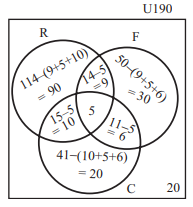The number of students who liked any one of the music = 90 + 9 + 30 + 10 + 5 + 6 + 20

= 170

(a) The number of students who didn’t like any 3 types of music = 190 – 170 = 20

(b) Number of students who liked any two types ony = 9 + 6 + 10 = 25

(c) Number of students who liked folk music but not rock music = 30 + 6 = 36

32. A function f : [-7, 6) → R is defined as follows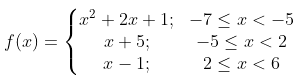Find:

(a) f(-7) – f(-3)

(b) [4f(-3) + 2f(4)]/ [f(-6) – 3f(1)]

Solution:

(a)

f(-7) = x2 + 2x + 1

= (-7)2 + 2(-7) + 1

= 49 – 14 + 1

= 36

f(-3) = x + 5 = -3 + 5 = 2

f(-7) – f(-3) = 36 – 2 = 34

(b)

f(4) = x – 1 = 4 – 1 = 3

f(-6) = x2 + 2x + 1

= (-6)2 + 2(-6) + 1

= 36 – 12 + 1

= 25

f(1) = 1 + 5 = 6

[4f(-3) + 2f(4)]/ [f(-6) – 3f(1)] = [4 × 2 + 2 × 3]/ [25 – 3 × 6]

= (8 + 6)/(25 – 18)

= 14/7

= 2

33. Find the three consecutive terms in an AP whose sum is 18 and the sum of their squares is 140.

Solution:

Let a – d, a, a + d be the three terms of an AP.

According to the given,

Sum of the three terms = 18

a – d + a + a + d = 18

3a = 18

a = 18/3

a = 6

Sum of square of three terms = 140

(a – d)2 + a2 + (a + d)2 = 140

a2 + d2 – 2ad + a2 + a2 + d2 + 2ad = 140

3(6)2 + 2d2 = 140

2d2 = 140 – 108

d2 = 32/2

d2 = 16

d = ±4

If a = 6 and d = 4, then the three terms are 2, 6, 10

If a = 6 and s = -4, then the three terms are 10, 6, 2.

34. Solve 3(2x + y) = 7xy; 3(x + 3y) = 11xy using elimination method.

Solution:

Given,

3(2x + y) = 7xy

6x + 3y = 7xy ….(i)

3(x + 3y) = 11xy

3x + 9y = 11xy ….(ii)

(i) × – (ii),

18x + 9y – (3x + 9y) = 21xy – 11xy

15x = 10xy

⇒ y = 15/10

⇒ y = 3/2

Substituting y = 3/2 in (i),

6x + 3(3/2) + 7x(3/2)

6x + (9/2) = 21x/2

(12x + 9)/2 = 21x/2

12x + 9 = 21x

⇒ 21x – 12x = 9

⇒ 9x = 9

⇒ x = 1

Also, if x = 0, then y = 0

Hence, the solution of the given system of equations is (1, 3/2) and (0, 0).

35. Find the square root of the polynomial 4 + 25x2 – 12x – 24x3 + 16x4 by division method.

Solution:

Given,

4 + 25x2 – 12x – 24x3 + 16x4

= 16x4 – 24x3 + 25x2 – 12x + 4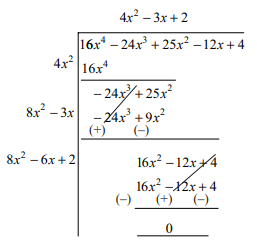Therefore, the square root of the polynomial = |4x2 – 3x + 2|

36. The difference of the squares of two positive numbers is 45. The square of the smaller number is four times the larger number. Find the numbers.

Solution:

Let x and y be the two numbers, where x > y.

According to the given,

x2 – y2 = 45 ….(i)

y2 = 4x ….(ii)

From (i) and (ii),

x2 – 4x – 45 = 0

x2 – 9x + 5x – 45 = 0

x(x – 9) + 5(x – 9) = 0

(x – 9)(x + 5) = 0

x = 9, x = -5

x cannot be negative.

Therefore, x = 9

y2 = 4(9)

y2 = 36

y = 6

Hence, the required numbers are 9 and 6.

37.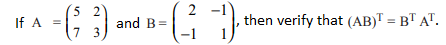Solution:

Given,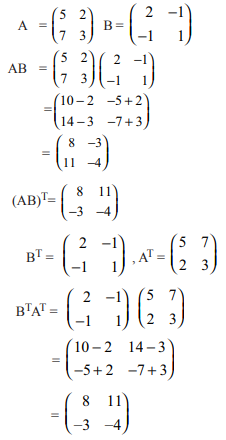Therefore, (AB)T = BTAT

38. Find the area of the quadrilateral whose vertices are (-3, 4), (-5, -6), (4, -1) and (1, 2).

Solution:

Given, vertices are (-3, 4), (-5, -6), (4, -1) and (1, 2).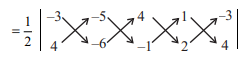= (1/2) |(18 + 5 + 8 + 4) – (20 – 24 – 1 – 6)|

= (1/2) |35 – (-51)|

= (1/2) |86|

= 43 sq.units

39. The midpoints D, E, F of the sides of a triangle ABC are (3, 4), (8, 9) and (6, 7) respectively. Find the vertices of the triangle.

Solution:

D, E, F are the midpoints of the sides AB, BC and AC of triangle ABC.

D = (3, 4)

E = (8, 9)

F = (6, 7)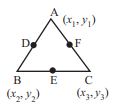Using the midpoint formula,

[(x1 + x2)/2, (y1 + y2)/2] = (3, 4)

x1 + x2 = 6 …..(i)

y1 + y2 = 8 …..(ii)

Similarly,

x2 + x3 = 16 ….(iii)

y2 + y3 = 18 ….(iv)

And

x1 + x3 = 12 ….(v)

y1 + y3 = 14 …..(vi)

2(x1 + x2 + x3) = 6 + 16 + 12

x1 + x2 + x3 = 34/2 = 17 …..(vii)

From (i), (iii), (v) and (vii),

x1 = 1, x2 = 5, x3 = 11

2(y1 + y2 + y3) = 8 + 18 + 14

y1 + y2 + y3 = 40/2 = 20 …..(viii)

From (ii), (iv), (vi) and (viiii),

y1 = 2, y2 = 6, y3 = 12

Hence, the coordinates of the vertices are A(1, 2), B(5, 6) and C(11, 12).

40. A lotus is 20 cm above the water surface in a pond and its stem is partly below the water surface. As the wind blew, the stem was pushed aside so that the lotus touched the water 40 cm away from the original position of the stem. How much of the stem was below the water surface originally?

Solution:

Let B is the lotus at a height of 20cm from the surface of water.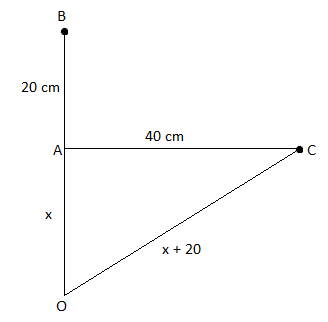OC = OA + AB = (x + 20) cm

AC = 40 cm

In right triangle OAC,

By Pythagoras theorem,

OC2 = OA2 + AC2

(x + 20)2 = x2 + (40)2

x2 + 40x + 400 = x2 + 1600

40x = 1600 – 400

40x = 1200

x = 1200/40

x = 30 cm

Hence, the stem was 30 cm below the water surface originally.

41. The angle of elevation of the top of a building from the foot of the tower is 30° and the angle of elevation of the top of the tower from the foot of the building is 60°. If the tower is 50 m high, find the height of the building.

Solution:

Let AB be the building and CD be the tower.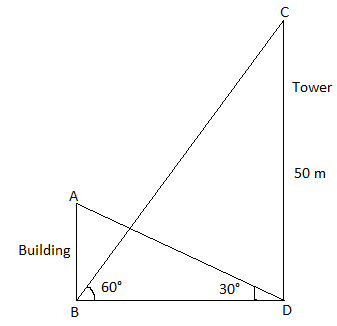In triangle CDB,

tan 60° = CD/BD

√3 = 50/BD

⇒ BD = 50/√3 m

In triangle ABD,

tan 30° = AB/BD

1/√3 = AB/BD

⇒ BD = AB√3

⇒ 50/√3 = AB√3

⇒ AB = 50/3

⇒ AB = 16.67 m

Therefore, the height of the building is 16.67 m.

42. The sum of the base radius and the height of a right circular solid cylinder is 37 cm. If the total surface area of the cylinder is 1628 sq.cm, then find the volume of the cylinder.

Solution:

Let r be the base radius and h be the height of the right circular cylinder.

Given,

r + h = 37 cm

Total surface area = 1628 sq.cm

2πr(r + h) = 1628

2 × (22/7) × r × 37 = 1628

r = (1628 × 7)/ (2 × 22 × 37)

r = 7 cm

Thus, h = 37 – r = 37 – 7 = 30 cm

Volume of the cylinder = πr2h

= (22/7) × 7 × 7 × 30

= 22 × 7 × 30

= 4620 cm3

43. For a collection of data, if ∑x = 35, n = 5, ∑(x – 9)2 = 82, then find ∑x2 and ∑(x – x bar)2.

Solution:

Given,

∑x = 35, n = 5, ∑(x – 9)2 = 82

x bar = ∑x/n = 35/5 = 7

∑(x – 9)2 = 82

∑(x2 – 18x + 81) = 82

∑x2 – 18∑x + 81∑1 = 82

∑x2 – 18(35) + 81(5) = 82

∑x2 – 630 + 405 = 82

∑x2 = 630 + 82 – 405

∑x2 = 307

Similarly,

∑(x – 9)2 = 82

∑(x – 7 – 2)2 = 82

∑[(x – 7) – 2]2 = 82

∑(x – 7)2 – 4∑(x – 7) + 4∑1 = 82

∑(x – x bar)2 – 4∑(x – x bar) + 4(5) = 82

∑(x – x bar)2 – 4(0) + 20 = 82

∑(x – x bar)2 = 82 – 20

∑(x – x bar)2 = 62

44. Two dice are rolled simultaneously. Find the probability that the sum of the numbers on the faces is neither divisible by 3 nor by 4.

Solution:

Total number of outcomes = n(S) = 36

Let A be the event that the sum of numbers on dice is divisible by 3.

A = {(1, 2), (2, 1), (2, 4), (4, 2), (1, 5), (5, 1), (3, 3), (3, 6), (6, 3), (4, 5), (5, 4), (6, 6)}

n(A) = 12

P(A) = n(A)/n(S) = 12/36

Let B be the event that the sum of numbers on dice is divisible by 4.

B = {(1, 3), (3, 1), (2, 2), (2, 6), (6, 2), (3, 5), (5, 3), (4, 4), (6, 6)}

n(B) = 9

P(B) = n(B)/n(S) = 9/36

A ⋂ B = {(6, 6)}

n(A ⋂ B) = 1

P(A ⋂ B) = 1/36

P(A ⋃ B) = P(A) + P(B) – P(A ⋂ B)

= (12/36) + (9/36) – (1/36)

= (12 + 9 – 1)/36

= 20/36

= 5/9

Probability that the sum of the numbers on the faces is neither divisible by 3 nor by 4 = 1 – P(A ⋃ B)

= 1 – (5/9)

= (9 – 5)/9

= 4/9

45. (a) The first term of a geometric series is 375 and the fourth term is 192. Find the common ratio and the sum of the first 14 terms.

Solution:

Given,

First term of GP = a = 375

Fourth term = t4 = arn – 1

ar3 = 192

r3 = 192/375

r3 = (4/5)3

r = 4/5

Sum of first n terms of GP

Sn = 1(1 – rn)/(1 – r)

Sum of the first 14 terms is

S14 = 375[1 – (4/5)14]/ [1 – (4/5)]

= 375 × 5 [1 – (4/5)14]

= 1875 [1 – (4/5)14]

OR

(b) Water in a cylindrical tank of diameter 4 m and height 10 m is released through a cylindrical pipe of diameter 10 cm at the rate of 2.5 km/hr. How much time will it take to empty half of the tank? (Assume that the tank is full of water to begin with)

Solution:

Given,

Diameter of tank = 4 m

Radius of the tank = R = 2 m

Height of the tank = H = 10 m

Diameter of pipe = 10 cm

Radius of pipe = r = 10/2 = 5 cm

r = 5/100 m = 1/20 m

Speed = 2.5 km/hr = 2500 m/hr

Let t be the time required to empty half of the tank.

Volume of water released through the pipe = 1/2(volume of tank)

πr2 × t × speed = (1/2)πR2H

(1/20)2 × t × 2500 = (1/2) × (2)2 × 10

t = (20 × 400)/2500

t = 3.2 hours = 3 hours 12 min

SECTION – IV

46. (a) Take a point which is 9 cm away from the centre of a circle of radius 3 cm, and draw two tangents to the circle from that point and calculate their lengths.

Solution: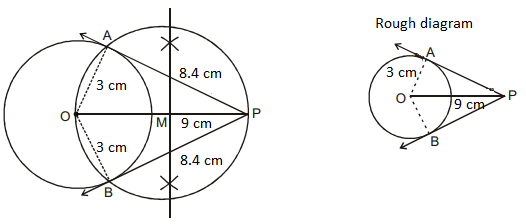Length of the tangents = 8.4 cm

OR

(b) Construct a cyclic quadrilateral PQRS with PQ = 4 cm, QR = 6 cm, PR = 7.5 cm, QS = 7 cm.

Solution: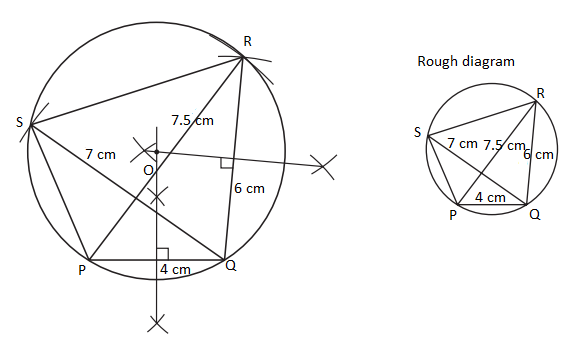47. (a) Draw the graph of y = x2 + 3x + 2 and use it to solve the equation x2 + 2x + 4 = 0.

Solution:

Given,

y = x2 + 3x + 2

 x -4 -3 -2 -1 0 1 2 3 x2 16 9 4 1 0 1 4 9 3x -12 -9 -6 -3 0 3 6 9 2 2 2 2 2 2 2 2 2 y 6 2 0 0 2 6 12 20

Now,

Subtracting x2 + 2x + 4 = 0 from y = x2 + 3x + 2

y – 0 = x2 + 3x + 2 – (x2 + 2x + 4)

y = x – 2

 x -2 0 1 2 y -4 -2 -1 0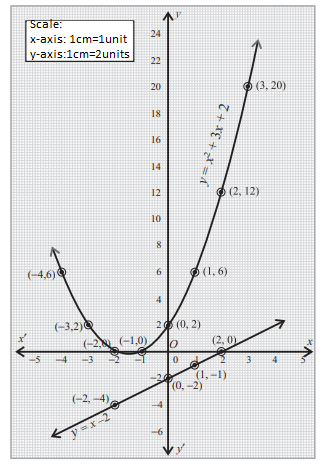From the graph, the solution set does not have real roots, i.e. imaginary.

OR

(b) The cost of milk per litre is Rs. 15. Draw the graph for the relation between the quantity and cost. Hence, find

(i) the proportionality constant

(ii) the cost of 3 litres of milk

Solution:

Let x be the quantity of milk (in litres) and y be the cost of milk (in Rs.).

 x 1 2 3 4 5 6 y 15 30 45 60 75 90

(i) The proportionality constant = k = 15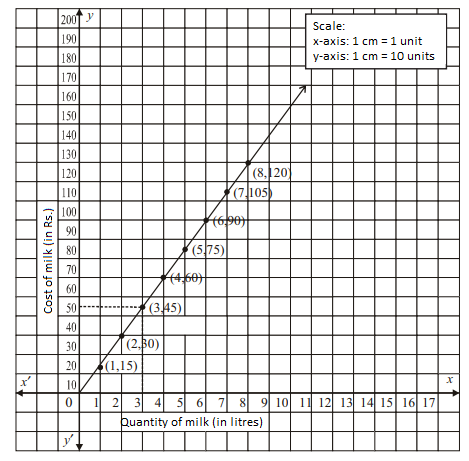(ii) The cost of 3 litres of milk = Rs. 45